## MIX10: A MATLAB TO X10 COMPILER FOR HIGH PERFORMANCE

by

School of Computer Science
McGill University, Montréal

Monday, April 14th 2014 A THESIS SUBMITTED TO THE FACULTY OF GRADUATE STUDIES AND RESEARCH
IN PARTIAL FULfiLLMENT OF THE REQUIREMENTS FOR THE DEGREE OF
MASTER OF SCIENCE Copyright © 2014 Vineet Kumar

Abstract

MATLAB is a popular dynamic array-based language commonly used by students, scientists and engineers who appreciate the interactive development style, the rich set of array operators, the extensive builtin library, and the fact that they do not have to declare static types. Even though these users like to program in MATLAB, their computations are often very compute-intensive and are better suited for emerging high performance computing systems. This thesis reports on MIX10, a source-to-source compiler that automatically translates MATLAB programs to X10, a language designed for “Performance and Productivity at Scale"; thus, helping scientific programmers make better use of high performance computing systems.

There is a large semantic gap between the array-based dynamically-typed nature of MATLAB and the object-oriented, statically-typed, and high-level array abstractions of X10. This thesis addresses the major challenges that must be overcome to produce sequential X10 code that is competitive with state-of-the-art static compilers for MATLAB which target more conventional imperative languages such as C and Fortran. Given that efficient basis, the thesis then provides a translation for the MATLAB parfor construct that leverages the powerful concurrency constructs in X10.

The MIX10 compiler has been implemented using the McLab compiler tools, is open source, and is available both for compiler researchers and end-user MATLAB programmers. We have used the implementation to perform many empirical measurements on a set of 17 MATLAB benchmarks. We show that our best MIX10-generated code is significantly faster than the de facto Mathworks’ MATLAB system, and that our results are competitive with state-of-the-art static compilers that target C and Fortran. We also show the importance of finding the correct approach to representing the arrays in X10, and the necessity of an IntegerOkay analysis that determines which double variables can be safely represented as integers. Finally, we show that our X10-based handling of the MATLAB parfor greatly outperforms the de facto MATLAB implementation.

Résumé

MATLAB est un langage de programmation dynamique, orienté-tableaux communément utilisé par les étudiants, les scientifiques et les ingénieurs qui apprécient son style de développement interactif, la richesse de ses opérateurs sur les tableaux, sa librairie impressionnante de fonctions de base et le fait qu’on aie pas à déclarer statiquement le type des variables. Bien que ces usagers apprécient MATLAB, leurs programmes nécessitent souvent des ressources de calcul importantes qui sont offertes par les nouveaux systèmes de haute performance. Cette thèse fait le rapport de MIX10, un compilateur source-à-source qui fait la traduction automatique de programmes MATLAB à X10, un langage construit pour "la performance et la productivité à grande échelle." Ainsi, MIX10 aide les programmeurs scientifiques à faire un meilleur usage des ressources des systèmes de calcul de haute performance.

Il y a un écart sémantique important entre le typage dynamique et le focus sur les tableaux de MATLAB et l’approche orientée-objet, le typage statique et les abstractions de haut niveau sur les tableaux de X10. Cette thèse discute des défis principaux qui doivent être surmontés afin de produire du code X10 séquentiel compétitif avec les meilleurs compilateurs statiques pour MATLAB qui traduisent vers des langages impératifs plus conventionnels, tels que C et Fortran. Fort de cette fondation efficace, cette thèse décrit ensuite la traduction de l’instruction parfor de MATLAB afin d’utiliser les opérations sophistiquées de traitement concurrent de X10.

Le compilateur MIX10 a été implémenté à l’aide de la suite d’outils de McLab, un projet libre de droits, disponible à la communauté de recherche ainsi qu’aux utilisateurs de MATLAB. Nous avons utilisé notre implémentation afin d’effectuer des mesures empiriques de performance sur un jeu de 17 programmes MATLAB. Nous démontrons que le code généré par MIX10 est considérablement plus rapide que le système MATLAB de Mathworks et que nos résultats sont compétitifs avec les meilleurs compilateurs statiques qui produisent du code C et Fortran. Nous montrons également l’importance d’une représentation appropriée des tableaux en X10 et la nécessité d’une analyse IntegerOkay qui permet de déterminer quelles variables de type réel (double) peuvent être correctement représentées par des entiers (int). Finalement, nous montrons que notre traitement de l’instruction parfor en X10 nous permet d’atteindre des vitesses d’exécution considérablement meilleures que dans MATLAB.

Acknowledgements

I am thankful to my supervisor, Laurie Hendren, whose constant encouragement and guidance not only helped me to understand compilers better, but also helped me become a better writer and a better presenter.

I would like to give a special thanks to David Grove for suggesting this line of research and in helping us understand key parts of the X10 language and implementation.

I am also thankful to the McLAB team for building an amazing framework for compiler research on MATLAB. In particular, I am thankful to Anton Dubrau (M.Sc.) for helping me understand the MCSAF and Tamer frameworks, and Xu Li (M.Sc.) for all the interesting discussions on how to statically compile MATLAB.

Finally, I would like to thank my parents and my brother, who made it possible for me to pursue my M.Sc. degree.

Apart from all the wonderful people around me, I am thankful to my computers, Antriksh, Heisenberg, and cougar.cs.mcgill.ca for tirelessly and reliably crunching numbers day in and day out.

This work was supported, in part, by the Natural Sciences and Engineering Research Council of Canada (NSERC).

Table of Contents

List of Figures

List of Tables

Chapter 1

Introduction

___________________________________________________________________________________

MATLAB is a popular numeric programming language, used by millions of scientists, engineers as well as students worldwide[Mol]. MATLAB programmers appreciate the high-level matrix operators, the fact that variables and types do not need to be declared, the large number of library and builtin functions available, and the interactive style of program development available through the IDE and the interpreter-style read-eval-print loop. However, even though MATLAB programmers appreciate all of the features that enable rapid prototyping, their applications are often quite compute intensive and time consuming. These applications could perform much more efficiently if they could be easily ported to a high performance computing system.

X10 [IBM12], on the other hand, is an object-oriented and statically-typed language which uses cilk-style arrays indexed by Point objects and rail-backed multidimensional arrays, and has been designed with well-defined semantics and high performance computing in mind. The X10 compiler can generate C++ or Java code and supports various communication interfaces including sockets and MPI for communication between nodes on a parallel computing system.

In this thesis we present MIX10, a source-to-source compiler that helps to bridge the gap between MATLAB, a language familiar to scientists, and X10, a language designed for high performance computing systems. MIX10 statically compiles MATLAB programs to X10 and thus allows scientists and engineers to write programs in MATLAB (or use old programs already written in MATLAB) and still get the benefits of high performance computing without having to learn a new language.Also, systems that use MATLAB for prototyping and C++ or Java for production, can benefit from MIX10 by quickly convert- ing MATLAB prototypes to C++ or Java programs via X10

On one hand, all the aforementioned characteristics of MATLAB make it a very user-friendly and thus a popular application to develop software among a non-programmer community. On the other hand, these same characteristics make MATLAB a difficult language to compile statically. Even the de facto standard, Mathworks’ implementation of MATLAB is essentially an interpreter with a JIT accelarator[The02] which is generally slower than statically compiled languages. GNU Octave, which is a popular open source alternative to MATLAB and is mostly compatible with MATLAB, introduced JIT compilation only in its most recent release 3.8 in March 2014. Before that it was implemented only as an interpreter[Oct]. Lack of formal language specification, unconventional semantics and closed source make it even harder to write a compiler for MATLAB. These are some of the challenges that MIX10 shares with other static compilers which convert MATLAB to C or Fortran. However, targeting X10 raises some significant new challenges. For example, X10 is an object-oriented, heap-based, language with varying levels of high-level abstractions for arrays and iterators of arrays. An open question was whether or not it was possible to generate X10 code that both maintains the MATLAB semantics, but also leads to code that is as efficient as state-of-the-art translators that target C and Fortran. Finding an effective and efficient translation from MATLAB to X10 was not obvious, and this thesis reports on the key problems we encountered and the solutions that we designed and implemented in our MIX10 system. By demonstrating that we can generate sequential X10 code that is as efficient as generated C or Fortran code, we then enabled the possibility of leveraging the high performance nature of X10’s parallel constructs. To demonstrate this, we exposed scalable concurrency in MATLAB via X10 and examined how to use X10 features to provide a good implementation for MATLAB’s parfor construct.

Built on top of McLAB static analysis framework[Doh11DH12b], MIX10, together with its set of reusable static analyses for performance optimization and extended support for MATLAB features, ultimately aims to provide MATLAB’s ease of use, sequential performance comparable to that provided by state-of-the art compilers targetting C and Fortran, to support parallel constructs like parfor and to expose scalable concurrency.

### 1.1 Contributions

The major contributions of this thesis are as follows:

Identifying key challenges:
We have identified the key challenges in performing a semantics-preserving efficient translation of MATLAB to X10.
Overall design of MIX10:
Building upon the McLAB frontend and analysis framework, we provide the design of the MIX10 source-to-source translator that includes a low-level X10 IR and a template-based specialization framework for handling builtin operations.
Techniques for efficient compilation of MATLAB arrays:
Arrays are the core of MATLAB. All data, including scalar values are represented as arrays in MATLAB. Efficient compilation of arrays is the key for good performance. X10 provides two types of array representations for multidimensional arrays: (1) Cilk-styled, region-based arrays and (2) rail-backed simple arrays. We compare and contrast these two array forms for a high performance computing language in context of being used as a target language and provide techniques to compile MATLAB arrays to two different representations of arrays provided by X10.
Builtin handling framework:
We have designed and implemented a template-based system that allows us to generate specialized X10 code for a collection of important MATLAB builtin operations.
Code generation strategies for the sequential core:
There are some very significant differences between the semantics of MATLAB and X10. A key difference is that MATLAB is dynamically-typed, whereas X10 is statically-typed. Furthermore, the type rules are quite different, which means that the generated X10 code must include the appropriate explicit type conversion rules, so as to match the MATLAB semantics. Other MATLAB features, such as multiple returns from functions, a non-standard semantics for for loops, and a very general range operator, must also be handled correctly.
Code generation for concurrency in MATLAB:
MIX10 not only supports all the key sequential constructs but also supports concurrency constructs like parfor and can handle vectorized instructions in a concurrent fashion. We have also introduced X10-like concurrency constructs in MATLAB via structured comments.
Safe use of integer variables:
Even after determining the correct use of X10 arrays, we were still faced with a performance gap between the code generated by the C/Fortran systems, and the generated X10 code generated by MIX10. Furthermore, we found that the MIX10 system using the X10 Java backend was often generating better code than with the X10 C++ backend, which was counter-intuitive.

We determined that a key remaining problem was overhead due to casting doubles to integers. This casting overhead was quite high, and was substantially higher for the C++ back-end than for the Java back-end (since the casting instructions are handled efficiently by the Java VM). This casting problem arises because the default data type for MATLAB variables is double - even variables used to iterate through arrays, and to index into arrays typically have double type, even though they hold integral values. To tackle this issue we developed an IntegerOkay analysis which determines which double variables can be safely converted to integer variables in the generated X10 code, hence greatly reducing the number of casting operations needed.

Open implementation:
We have implemented the MIX10 compiler over various MATLAB compiler tools provided by the McLAB toolkit. In the process we also implemented some enhancements to these existing tools. Our implementation is extensible and allows for others to make further improvements to the generated X10 code, or to reuse the analyses to generate code for other target languages.
Experimental evaluation:
We have experimented with our MIX10 implementation on a set of benchmarks. Our results show that our generated code is almost an order of magnitude faster than the Mathworks’ standard JIT-based system, and is competitive with other state-of-the-art tools that generate C/Fortran. Our more detailed results show the importance of using our customized and optimized X10 array representations, and we demonstrate the effectiveness of the IntegerOkay analysis. Finally, we show that our X10-based treatment of parfor significantly outperforms MATLAB on our benchmarks.

### 1.2 Thesis Outline

This thesis is divided into 11 chapters, including this one and is structured as follows.

Chapter 2 provides an introduction to the X10 language and describes how it compares to MATLAB from the point of view of language design. Chapter 3 gives a description of various existing MATLAB compiler tools upon which MIX10 is implemented, presents a high-level design of MIX10, and explains the design and need of MIX10 IR. In Chapter 4 we compare the two kinds of arrays provided by X10, identify when each of them must be used in the generated code, identify and address challenges involved in efficient compilation of MATLAB arrays. Chapter 5 describes the builtin handling framework used by MIX10 to generate specialized code for MATLAB builtins used in the source program. Chapter 6 gives a description of efficient and effective code generation strategies for the core sequential constructs of MATLAB. A description of code generation for the MATLAB parfor construct is provided in Chapter 7, which also describes our strategy to introduce concurrency constructs in MATLAB. In Chapter 8 we provide a description of the IntegerOkay analysis to identify variables that are safe to be declared as Long type. Chapter 9 provides performance results for code generated using MIX10 for a suite of benchmarks. It gives a comparison between performance achieved by MIX10 generated code and that generated by the MATLAB coder and MC2FOR compilers. Chapter 10 provides an overview of related work and Chapter 11 concludes and outlines possible future work. Chapter 2

Introduction to the X10 Programming Language

___________________________________________________________________________________

In this chapter, we describe key X10 semantics and features to help readers unfamiliar with X10 to have a better understanding of the MIX10 compiler.1

X10 is an award winning open-source programming language being developed by IBM Research. The goal of the X10 project is to provide a productive and scalable programming model for the new-age high performance computing architectures ranging from multi-core processors to clusters and supercomputers [IBM12].

X10, like Java, is a class-based, strongly-typed, garbage-collected and object-oriented language. It uses Asynchronous Partitioned Global Address Space (APGAS) model to support concurrency and distribution [SBP+12]. The X10 compiler has a native backend that compiles X10 programs to C++ and a managed backend that compiles X10 programs to Java.

### 2.1 Overview of X10’s Key Sequential Features

X10’s sequential core is a container-based object-oriented language that is very similar to that of Java or C++ [SBP+12]. An X10 program consists of a collection of classes, structs or interfaces, which are the top-level compilation units. X10’s sequential constructs like if-else statements, for loops, while loops, switch statements, and exception handling constructs throw and trycatch are also same as those in Java. X10 provides both, implicit coercions and explicit conversions on types, and both can be defined on user-defined types. The as operator is used to perform explicit type conversions; for example, x as Long{self != 0} converts x to type Long and throws a runtime exception if its value is zero. Multi-dimensional arrays in X10 are provided as user-defined abstractions on top of x10.lang.Rail, an intrinsic one-dimensional array analogous to one-dimensional arrays in languages like C or Java. Two families of multi-dimensional array abstractions are provided: simple arrays, which provide a restricted but efficient implementation, and region arrays which provide a flexible and dynamic implementation but are not as efficient as simple arrays. Listing 2.1 shows a sequential X10 program that calculates the value of π using the Monte Carlo method.2 It highlights important sequential and object-oriented features of X10 detailed in the following subsections. It reads an argument from the console in an intrinsic 1 dimensions array(Rail) of String and converts it to a Long value N. It then generates two random numbers and performs computations on them in a loop from 1 to N. Finally, it calculates the value of π and displays the result on the console.

X10’s syntax is similar to that of Java. The object-oriented semantics are also similar to that of Java. Mutable and immutable variables are denoted by keywords var and val respectively. for loops can iterate over a range of values. For e.g. in listing 2.1, the for loop iterates over a LongRange of 1 to N. A LongRange represents a range of Long type integers.

import x10.util.Random;

public class SeqPi {
public static def main(args:Rail[String]) {
val N = Long.parse(args(0));
var result:Double = 0;
val rand = new Random();
for(1..N) {
val x = rand.nextDouble();
val y = rand.nextDouble();
if(x*x + y*y <= 1) result++;
}
val pi = 4*result/N;
Console.OUT.println(~The value of pi is ~ + pi);
}
}
Listing 2.1: Sequential X10 program to calculate value of π using Monte Carlo method

#### 2.1.1 Object-oriented Features

A program consists of a collection of top-level units, where a unit is either a class, a struct or an interface. A program can contain multiple units, however only one unit can be made public and its name must be same as that of the program file. Similar to Java, access to these top-level units is controlled by packages. Below is a description of the core object-oriented constructs in X10:

Class
A class is a basic bundle of data and code. It consists of zero or more members namely fields, methods, constructors, and member classes and interfaces [IBM13b]. It also specifies the name of its superclass, if any and of the interfaces it implements.
Fields
A field is a data item that belongs to a class. It can be mutable (specified by the keyword var) or immutable (specified by the keyword val). The type of a mutable field must be always be specified, however the type of an immutable field may be omitted if it’s declaration specifies an initializer. Fields are by default instance fields unless marked with the static keyword. Instance fields are inherited by subclasses, however subclasses can shadow inherited fields, in which case the value of the shadowed field can be accessed by using the qualifier super.
Methods
A method is a named piece of code that takes zero or more parameters and returns zero or one values. The type of a method is the type of the return value or void if it does not return a value. If the return type of a method is not provided by the programmer, X10 infers it as the least upper bound of the types of all expressions e in the method where the body of the method contains the statement return e. A method may have a type parameter that makes it type generic. An optional method guard can be used to specify constraints. All methods in a class must have a unique signature which consists of its name and types of its arguments.

Methods may be inherited. Methods defined in the superclass are available in the subclasses, unless overridden by another method with same signature. Method overloading allows programmers to define multiple methods with same name as long as they have different signatures. Methods can be access-controlled to be private, protected or public; private methods can only be accessed by other methods in the same class, protected methods can be accessed in the same class or its subclasses, and public methods can be accessed from any code. By default, all methods are package protected which means they can be accessed from any code in the same package.

Methods with the same name as that of the containing class are called constructors. They are used to instantiate a class.

Structs
A struct is just like a class, except that it does not support inheritance and may not be recursive. This allows structs to be implemented as header-less objects, which means that unlike a class, a struct can be represented by only as much memory as is necessary to represent its fields and with its methods compiled to static methods. It does not contain a header that contains data to represent meta-information about the object. The current version of X10 (version 2.4) does not support mutability and references to structs, which means that there is no syntax to update the fields of a struct and structs are always passed by value.
Function literals
X10 supports first-class functions. A function consists of a parameter list, followed optionally by a return type, followed by =>, followed by the body (an expression). For example, (i:Int, j:Int) => (i<j ? foo(i) : foo(j)), is a function that takes parameters i and j and returns foo(i) if i<j and foo(j) otherwise. A function can access immutable variables defined outside the body.

#### 2.1.2 Statements

X10 provides all the standard statements similar to Java. Assignment, if - else and while loop statements are identical to those in Java.

for loops in X10 are more advanced and apart from the standard C-like for loop, X10 provides three different kinds of for loops:

enhanced for loops take an index specifier of the form i in r, where r is any value that implements x10.lang.Iterable[T] for some type T. Code listing 2.2 below shows an example of this kind of for loops:
def sum(a:Rail[Long]):Long{
var result:Long = 0;
for (i in a){
result += i;
}
return result;
}
Listing 2.2: Example of enhanced for loop
for loops over LongRange iterate over all the values enumerated by a LongRange(a range of consecutive Long type values). A LongRange is instantiated by an expression like e1..e2 and enumerates all the integer values from a to b (inclusive) where e1 evaluates to a and e2 evaluates to b. Listing 2.3 below shows an example of a for loop that uses LongRange:
def sum(N:Long):Long{
var result:Long = 0;
for (i in 0..N){
result += i;
}
return result;
}
Listing 2.3: Example of for loop over LongRange
for loops over Region allow to iterate over multiple dimensions simultaneously. A Region is a data structure that represents a set of points in multiple dimensions. For instance, a Region instantiated by the expression Region.make(0..5,1..6) creates a 2-dimensional region of points (x,y) where x ranges over 0..5 and y over 1..6. The natural order of iteration is lexicographic. Listing 2.4 below shows an example that calculates the sum of coordinates of all points in a given rectangle:
def sum(M:Long, N:Long):Long{
var result:Long = 0;
val R:Region = x10.regionarray.Region.make(0..M,0..N);
for ([x,y] in R){
result += x+y;
}
return result;
}
Listing 2.4: Example of for loop over a 2-D Region

#### 2.1.3 Arrays

In order to understand the challenges of translating MATLAB to X10, one must understand the different flavours and functionality of X10 arrays.

At the lowest level of abstraction, X10 provides an intrinsic one-dimensional fixed-size array called Rail which is indexed by a Long type value starting at 0. This is the X10 counterpart of built-in arrays in languages like C or Java. In addition, X10 provides two types of more sophisticated array abstractions in packages, x10.array and x10.regionarray.

Rail-backed Simple arrays
are a high-performance abstraction for multidimensional arrays in X10 that support only rectangular dense arrays with zero-based indexing. Also, they support only up to three dimensions (specified statically) and row-major ordering. These restrictions allow effective optimizations on indexing operations on the underlying Rail. Essentially, these multidimensional arrays map to a Rail of size equal to number of elements in the array, in a row-major order.
Region arrays
are much more flexible. A region is a set of points of the same rank, where Points are the indexing units for arrays. Points are represented as n-dimensional tuples of integer values. The rank of a point defines the dimensionality of the array it indexes. The rank of a region is the rank of its underlying points. Regions provide flexibility of shape and indexing. Region arrays are just a set of elements with each element mapped to a unique point in the underlying region. The dynamicity of these arrays come at the cost of performance.

Both types of arrays also support distribution across places. A place is one of the central innovations in X10, which permits the programmer to deal with notions of locality. places are discussed in more detail in section 2.2.4

#### 2.1.4 Types

X10 is a statically type-checked language: Every variable and expression has a type that is known at compile-time and the compiler checks that the operations performed on an expression are permitted by the type of that expression. The name c of a class or an interface is the most basic form of type in X10. There are no primitive types.

X10 also allows type definitions, that allow a simple name to be supplied for a complicated type, and for type aliases to be defined. For example, a type definition like public static type bool(b:Boolean) = Boolean{self=b} allows the use of expression bool(true) as a shorthand for type Boolean{self=true}.

Generic types
X10’s generic types allow classes and interfaces to be declared parameterized by types. They allow the code for a class to be instantiated unbounded number of times, for different concrete types, in a type-safe fashion. For instance, the listing 2.5 below shows a class List[T], parameterized by type T, that can be replaced by a concrete type like Int at the time of instantiation (var l:List[Int] = new List[Int](item)).
class List[T]{
var item:T;
var tail:List[T]=null;
def this(t:T){
item=t;
}
}

X10 types are available at runtime, unlike Java(which erases them).

Constrained types
X10 allows the programmer to define Boolean expressions (restricted) constraints on a type [T]. For example, a variable of constrained type Long{self != 0} is of type Long and has a constraint that it can hold a value only if it is not equal to 0 and throws a runtime error if the constraint is not satisfied. The permitted constraints include the predicates == and !=. These predicates may be applied to constraint terms. A constraint term is either a final variable visible at the point of definition of the constraint, or the special variable self or of the form t.f where f names a field, and t is (recursively) a constraint term.

### 2.2 Overview of X10’s Concurrency Features

X10 is a high performance language that aims at providing productivity to the programmer. To achieve that goal, it provides a simple yet powerful concurrency model that provides four concurrency constructs that abstract away the low-level details of parallel programming from the programmer, without compromising on performance. X10’s concurrency model is based on the Asynchronous Partitioned Global Address Space (APGAS) model [IBM13a]. The APGAS model has a concept of global address space that allows a task in X10 to refer to any object (local or remote). However, a task may operate only on an object that resides in its partition of the address space (local memory). Each task, called an activity, runs asynchronously parallel to each other. A logical processing unit in X10 is called a place. Each place can run multiple activities. There are four types of concurrency constructs provided by X10 [IBM13b], as described in the following sections:

#### 2.2.1 Async

The fundamental concurrency construct in X10 is async. The statement async S creates a new activity to execute S and returns immediately. The current activity and the “forked" activity execute asynchronously parallel to each other and have access to the same heap of objects as the current activity. They communicate with each other by reading and writing shared variables. There is no restriction on statement S in the sense that it can contain any other constructs (including async). S is also permitted to refer to any immutable variable defined in lexically enclosing scope.

An activty is the fundamental unit of execution in X10. It may be thought of as a very light-weight thread of execution. Each activity has its own control stack and may invoke recursive method calls. Unlike Java threads, activities in X10 are unnamed. Activities cannot be aborted or interrupted once they are in flight. They must proceed to completion, either finishing correctly or by throwing an exception. An activity created by async S is said to be locally terminated if S has terminated. It is said to be globally terminated if it has terminated locally and all activities spawned by it recursively, have themselves globally terminated.

#### 2.2.2 Finish

Global termination of an activity can be converted to local termination by using the finish construct. This is necessary when the programmer needs to be sure that a statement S and all the activities spawned transitively by S have terminated before execution of the next statement begins. For instance in the listing 2.5 below, the use of finish ensures that the Console.OUT.println(~a(1) = ~ + a(1)); statement is executed only after all the asynchronously executing operations (async a(i) *= 2; have completed.

//...
//Create a Rail of size 10, with ith element initialized to i
val a:Rail[Long] = new Rail[Long](10,(i:Long)=>i);
finish for (i in 0..9){
//asynchronously double every value in the Rail
async a(i) *= 2;
}
Console.OUT.println(~a(1) = ~ + a(1));
//...
Listing 2.5: Example use of finish construct

#### 2.2.3 Atomic

The statement atomic S ensures that the statement S is executed in a single step with respect to all other activities in the system. When S is being executed in one activity all other activities containing s are suspended. However, the atomic statement S must be sequential (it should not contain an async or finish staement), non-blocking (it should not contain a blocking construct like when, explained below) and local (in this context, local means place-local, that is, it must not contain an at construct, explained in Sec. 2.2.4). Consider the code fragment in listing 2.6. It asynchronously adds Long values to a linked-list list and simultaneously holds the size of the list in a variable size. The use of atomic guarantees that no other operation, in any activity, is executed in between (or simultaneously with) these two operations, which is necessary to ensure correctness of the program.

//...
finish for (i in 0..10){
async add(i);
}
//...
def add(x:Long){
atomic {
this.list.add(x);
this.size = this.size + 1;
}
}
//...
Listing 2.6: Example use of atomic construct

Note that, atomic is a syntactic sugar for the construct when (c) , which is the conditional atomic statement based on binary condition (c). Statement when (c) S executes statement S atomically only when c evaluates to true; if it is false, the execution blocks waiting for c to be true. Condition c must be sequential, non-blocking and local.

#### 2.2.4 At

A place in X10 is the fundamental processing unit. It is a collection of data and activities that operate on that data. A program is run on a fixed number of places. The binding of places to hardware resources (e.g. nodes in a cluster, accelerators) is provided externally by a configuration file, independent of the program.

The at construct provides a place-shifting operation, that is used to force execution of a statement or an expression at a particular place. An activity executing at (p) S suspends execution at the current place; The object graph G at the current place whose roots are all the variables V used in S is serialized, and transmitted to place p, deserialized (creating a graph Gisomorphic to G), an environment is created with the variables V bound to the corresponding roots in G, and S executed at p in this environment. On local termination of S, computation resumes after at (p) S in the original location. The object graph is not automatically transferred back to the originating place when S terminates: any updates made to objects copied by an at will not be reflected in the original object graph.

### 2.3 Overview of X10’s Implementation and Runtime

In order to understand the compilation flow of the MIX10 compiler and enhancements made to the X10 compiler for efficient use of X10 as a target language for MATLAB, it is important to understand the design of the X10 compiler and its runtime environment.

#### 2.3.1 X10 Implementation

X10 is implemented as a source-to-source compiler that translates X10 programs to either C++ or Java. This allows X10 to achieve critical portability, performance and interoperability objectives. The generated C++ or Java program is, in turn, compiled by the platform C++ compiler to an executable or to class files by a Java compiler. The C++ backend is referred to as Native X10 and the Java backend is called Managed X10.

The source-to-source compilation approach in X10 provides three main advantages: (1) It makes X10 available for a wide range of platforms; (2) It takes advantage of the underlying classical and platform-specific optimizations in C++ or Java compilers, while the X10 implementation includes only X10 specific optimizations; and (3) It allows programmers to take advantage of the existing C++ and Java libraries.

Figure 2.1 shows the overall architecture of the X10 compiler [IBM13b].Figure 2.1: Architecture of the X10 compiler

#### 2.3.2 X10 Runtime

Figure 2.2 shows the major components of the X10 runtime and their relative hierarchy [IBM13b].

The runtime bridges the gap between application program and the low-level network transport system and the operating system. X10RT, which is the lowest layer of the X10 runtime, provides abstraction and unification of the functionalities provided by various network layers.

The X10 Language Native Runtime provides implementation of the sequential core of the language. It is implemented in C++ for native X10 and Java for Managed X10.

XRX Runtime, the X10 runtime in X10 is the core of the X10 runtime system. It provides implementation for the primitive X10 constructs for concurrency and distribution (async, finish, atomic and at). It is primarily written in X10 over a set of low-level APIs that provide a platform-independent view of processes, threads, synchronization mechanisms and inter-process communication.

At the top of the X10 runtime system, is a set of core class libraries that provide fundamental data types, basic collections, and key APIs for concurrency and distribution.Figure 2.2: Architecture of the X10 runtime

Chapter 3

Background and High-level Design of MIX10

___________________________________________________________________________________

### 3.1 Background

MIX10 is implemented on top of several existing MATLAB compiler tools. The overall structure is given in Figure 3.1, where the new parts are indicated by the shaded boxes, and future work is indicated by dashed boxes.Figure 3.1: Overview of MIX10 structure

As illustrated at the top of the figure, a MATLAB programmer only needs to provide an entry-point MATLAB function (called myprog.m in this example), plus a collection of other MATLAB functions and libraries (directories of functions) which may be called, directly or indirectly, by the entry point. The programmer may also specify the types and/or shapes of the input parameters to the entry-point function. As shown at the bottom of the figure, our MIX10 compiler automatically produces a collection of X10 output files which contain the generated X10 code for all reachable MATLAB functions, plus one X10 file called mix10.x10 which contains generated and specialized X10 code for the required builtin MATLAB functions. Thus, from the MATLAB programmer’s point of view, the MIX10 compiler is quite simple to use.

MATLAB is actually quite a complicated language to compile, starting with its rather unusual syntax, which cannot be parsed with standard LALR techniques. There are several issues that must be dealt with including distinguishing places where white space and new line characters have syntactic meaning, and filling in missing end keywords, which are sometimes optional. The McLAB front-end handles the parsing of MATLAB through a two step process. There is a pre-processing step which translates MATLAB programs to a cleaner subset, called Natlab, which has a grammar that can be expressed cleanly for a LALR parser. The McLAB front-end delivers a high-level AST based on this cleaner grammar.

After parsing, the next major phase of MIX10 uses the MCSAF framework [DH12aDoh11] to disambiguate identifiers using kind analysis [DHR11], which determines if an identifier refers to a variable or a named function. This is required because the syntax of MATLAB does not distinguish between variables and functions. For example, the expression a(i) could refer to four different computations, a could be an array or a function, and i could refer to the builtin function for the imaginary value i, or it could refer to a variable i. The MCSAF framework also simplifies the AST, producing a lower-level AST which is more amenable to subsequent analysis.

The next major phase is the Tamer [DH12b], which is a key component for any tool which statically compiles MATLAB. The Tamer generates an even more defined AST called Tamer IR, as well as performing key interprocedural analyses to determine both the call graph and an estimate of the base type and shape of each variable, at each program point. The call graph is needed to determine which files (functions) need to be compiled, and the type and shape information is very important for generating reasonable code when the target language is statically typed, as is the case for X10.

The Tamer also provides an extensible interprocedural value analysis and an interprocedural analysis framework that extends the intraprocedural framework provided by MCSAF. Any static backend will use the standard results of the Tamer, but is also likely to implement some target-language-specific analyses which estimate properties useful for generating code in a specific target language. Currently, we have implemented two analyses : (1) An analysis for determining if a MATLAB variable is real or complex to enable support for complex numbers in MIX10 and other MATLAB compilers based on McLAB; and (2) IntegerOkay analysis to identify which variables can be safely declared to be of an integer type (Int or Long) instead of the default type Double.

For the purposes of MIX10, the output of the Tamer is a low-level, well-structured AST, which along with key analysis information about the call graph, the types and shapes of variables, and X10-specific information. These Tamer outputs are provided to the code generator, which generates X10 code, and which is the main focus of this thesis.

The X10 source code generator actually gets inputs from two places. It uses the Tamer IR it receives from the the Tamer to drive the code generation, but for expressions referring to built-in MATLAB functions it interacts with the Built-in Handler which used the built-in template file we provide. We describe the functioning of the built-in handler in Chapter 5.

Chapter 4 concentrates on generating efficient code for MATLAB arrays. Chapter 6 describes the code generation strategy for the sequential core of MATLAB, while Chapter 7 describes our strategy to generate parallel X10 code for MATLAB parfor construct, and introducing X10 like concurrency constructs in MATLAB. The focus of this thesis is to address challenges in generating efficient X10 code whose performance is comparable to state-of-the-art tools that generate more traditional imperative languages like C and Fortran.

### 3.2 High-level Design of the MIX10 Compiler

The MIX10 code generator is the key component which makes the translation from the Tamer IR, which is based on MATLAB programming constructs and semantics, to X10. The overall structure of the MIX10 code generator is given in Figure 3.2.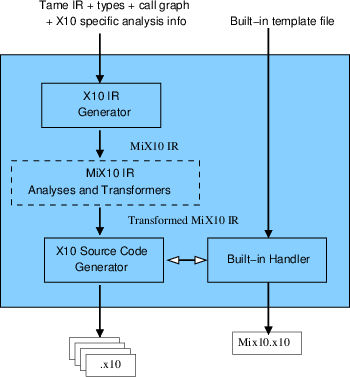Figure 3.2: Structure of the MiX10 code generator

The input to to MIX10 compiler is the call graph generated by Tamer and the Tame IR annotated with the necessary analysis information like shape, iscomplex, and type and IntegerOkay. Rather than do a direct code generation to X10 source code, MIX10 translates the Tamer IR to MIX10 IR, a general and extensible IR, designed by us, to represent X10. This translation is done by the X10 IR generator module of MIX10. Finally, with the inputs from the builtin handler and the X10 IR generator (after the X10 specific analyses and transformations have been done), the X10 source code generator generates the resultatnt X10 source code.

#### 3.2.1 The MIX10 Intermediate Representation

The MIX10 IR is a low-level, three address like intermediate representation that is similar in design to the Tamer IR and abstracts the X10 constructs while capturing the static information required to generate the X10 source code. We have implemented the IR using JastAdd [EH04jas], which allows us to easily add new AST nodes by simply extending the JastAdd specification grammar.

There are three important reasons to use an IR, instead of directly generating the X10 code:

• There are potentially two places that optimizations and transformations may happen: either at the Tamer IR level or at the MIX10 IR level. It is our intent to put any analysis or transformation that is not X10-specific into the Tamer IR, so that other back-ends can benefit from those improvements. However, optimizations and transformations that are specific to X10 programming constructs (such as points and regions) and semantics will need to be done on the MIX10 IR. Although we currently do not transform the MIX10 IR very much, the ultimate goal is to support a variety of analyses and transformations that can be used to: (1) produce more efficient X10 code, and (2) produce more readable X10 code.
• There are X10 constructs that can be pretty printed to be of different kinds, for example the X10 arrays, which can either be simple arrays, region arrays or specialized region arrays, and X10 for loop which can either be C-like for loop or an iterator over a LongRange (used in generated code for parfor loop). It allows to abstract the vital information for a construct while leaving the actual syntax to the source code generator. This makes it easier to add X10 specific analyses and transformations, and makes it easy to update the compiler whenever a new or improved variation of a construct is added.
• MIX10 IR can also be used as a convenient place to insert instrumentation code for the generated X10 code.

Appendix B provides the JastAdd implementation of the MIX10 IR grammar. Chapter 4

Techniques for Efficient Compilation of MATLAB Arrays

___________________________________________________________________________________

Arrays are the core of the MATLAB programming language. Every value in MATLAB is a Matrix and has an associated array shape. Even scalar values are represented as 1×1 arrays. Most of the data read and write operations involve accessing individual or a set of array elements. Given the central role of arrays in MATLAB, it is of utmost importance for our MIX10 compiler to find effective and efficient translations to X10 arrays.

Given the shape information provided by the shape analysis engine [LH14] built into the McLAB analysis framework [DH12aDoh11DH12b], it was not hard to compile MATLAB arrays to X10. However, to generate X10 code whose performance would be competitive to the generated C code (via MATLAB coder) and the generated Fortran code (via the MC2FOR compiler), was not straightforward, and required deeper understanding of the X10 array system and careful handling of several features of the MATLAB arrays.

### 4.1 Simple Arrays or Region Arrays

As described in Section 2.1.3, X10 provides two higher level abstractions for arrays, simple arrays, a high performance but rigid abstraction, and region arrays, a flexible abstraction but not as efficient as the simple arrays. In order to achieve more efficiency, our strategy is to use the simple arrays whenever possible, and to fall back to the region arrays when necessary. Note that it is possible to force the MIX10 compiler to use region arrays via a switch, for experimentation purposes.

#### 4.1.1 Compilation to Simple Arrays

In dealing with the simple rail-backed arrays, there were two important challenges. First, we needed to determine when it is safe to use the simple rail-backed arrays, and second, we needed an implementation of simple rail-backed arrays that handles the column-major, 1-indexing, and linearization operations required by MATLAB. When to use simple rail-backed arrays: After the shape analysis of the source MATLAB program, if shapes of all the arrays in the program: (1) are known statically, (2) are supported by the X10 implementation of simple arrays and (3) the dimensionality of the shapes remain same at all points in the program; then MIX10 generates X10 code that uses simple arrays. Column-major indexing: In order to make X10 simple arrays more compatible with MATLAB, we modified the implementation of the Array_2 and Array_3 classes in x10.array package to use column-major ordering instead of the default row-major ordering when linearizing multidimensional arrays to the backing Rail storage.1 1http://www.sable.mcgill.ca/mclab/mix10/x10_update/ Since MATLAB uses column-major ordering to linearize arrays, this modification also makes it trivial to support linear indexing operations in MATLAB. 2 2http://www.mathworks.com/help/matlab/math/matrix-indexing.html MATLAB naturally supports linear indexing for individual element access. More precisely, if the number of subscripts in an array access is less than the number of dimensions of the array, the last subscript is linearly indexed over the remaining number of dimensions in a column-major fashion. Our modification to use column-major ordering for the backing Rail make it easier and more efficient to support linear indexing by allowing direct access to the underlying Rail at the calculated linear offset.

Given that we can determine when it is safe to use the simple rail-backed arrays, and our improved X10 implementation of them, we then designed the appropriate translations from MATLAB to X10, for array construction, array accesses for both individual elements and ranges. Given the number of dimensions and the size of each dimension, it is easy to construct a simple array. For example a two-dimensional array A of type T and shape m×n can be constructed using a statement like val A:Array_2[T] = new Array_2[T](m,n);. Additional arguments can be passed to the constructor to initialize the array. Another important thing to note is that MATLAB allows the use of keyword end or an expression involving end (like end-1) as a subscript. end denotes the highest index in that dimension. If the highest index is not known the numElems_i property of the simple arrays is used to get the number of elements in the ith dimension of the array.

#### 4.1.2 Compilation to Region Arrays

With MATLAB’s dynamic nature and unconventional semantics, it is not always possible to statically determine the shape of an arrays accurately. Luckily, with some thought to a proper translation, X10’s region arrays are flexible enough to support MATLAB’s “wild" arrays. Also, since Point objects can be a set of arbitrary integers, there is no restriction on the starting index of the arrays. Region arrays can easily use one-based indexing. Array construction: Array construction for region arrays involves creating a region over a set of points (or index objects) and assigning it to an array. Regions of arbitrary ranks can be created dynamically. For example, consider the following MATLAB code snippet:

function[x] = foo(a)
t = bar(a);
x = t;
...
end

function[y] = bar(a)
if (a == 3)
y = zeros(a,a+1,a+2,a+3);
else
y = zeros(a,a+1,a+2);
end
end

In this code, the number of dimensions of array t and hence array x cannot be determined statically at compile-time. In such case, it is not possible to generate X10 code that uses simple arrays, however, it can still be compiled to the following X10 code for function foo().

static def foo(a: Double){
val t: Array[Double] =
new Array[Double](bar(a));
val x: Array[Double] =
new Array[Double](t);
...
return x;
}
static def bar(a:Double){
var y:Array[Double]=null;
if (a == 3) {
y = new Array[Double]
(Mix10.zeros(a,a+1,a+2,a+3));
}
else {
y = new Array[Double]
(Mix10.zeros(a,a+1,a+2));
}
return y;
}

In this generated X10 code, t is an array of type Double which can be created by copying from another array returned by bar(a) without knowing the shape of the returned array. Array access: Subscripting operations to access individual elements are mapped to X10’s region array subscripting operation. If the rank of array is 4 or less, it is subscripted directly by integers corresponding to subscripts in MATLAB otherwise we create a Point object from these integer values and use it to subscript the array. In case an expression involving end is used for indexing and the complete shape information is not available, method max(Long i), provided by the Region class is used, allowing to determine the highest index for a particular dimension at runtime. Rank specialization: Although region arrays can be used with minimal compile-time information, providing additional static information can improve performance of the resultant code by eliminating run-time checks involving provided information. One of the key specializations that we introduced with use of region arrays is to specify the rank of an array in its declaration, whenever it is known statically. For example if rank of an array A of type T is known to be two, it can be declared as val A:Array[T](2);. This specialization provided better performance compared to unspecialized code as shown in section 9.6.

### 4.2 Handling the Colon Expression

MATLAB allows the use of an expression such as a:b (or colon(a,b)) to create a vector of integers [a, a+1, a+2, ... b]. In another form, an expression like a:i:b can be used to specify an integer interval of size i between the elements of the resulting vector. Use of a colon expression for array subscripting takes all the elements of the array for which the subscript in a particular dimension is in the vector created by the colon expression in that dimension.3 Consider the following MATLAB code:

function [x] = crazyArray(a)
y = ones(3,4,5);
x = y(1,2:3,:);
end

Here y is a three-dimensional array of shape 3×4×5 and x is a sub-array of y of shape 1×2×5. Such array accesses can be handled by simply calling the getSubArray[T]() that we have implemented in a Helper class provided with the generated code. The generated X10 code with simple array for this example is as follows:

static def crazyArray (a: Double){
val y: Array_3[Double] = new Array_3[Double](Mix10.ones(3, 4, 5));
val mc_t0: Array_1[Double] = new Array_1[Double](Mix10.colon(2, 3));
var x: Array_3[Double];
x = new Array_3[Double](Helper.getSubArray(1, 1, mc_t0(0), mc_t0(1), 1, 5, y)) ;
return x;
}

The colon operator can also be used on the left hand side for an array set operation that updates multiple values of the array. For example, in the MATLAB statement x(:,4) = y;, all the values of the fourth column of x will be set to y if y is a scalar or to corresponding values of y if y is a column vector with length equal to the size of first dimension of x. To handle this kind of operation we have implemented another helper method, setSubArray(). This method takes as input, the target array, the bounds on each dimension, and the source array. x(:,4) = y; will be translated by MIX10 to x = Helper.setSubArray(x, 1, x.numElems_1, 4, 4, y);

We have implemented overloaded versions of the getSubArray() and the setSubArray() methods for arrays of different dimensions. For region arrays, we provide the same methods that operate on region arrays in a different version of the Helper class. MIX10 provides the correct version of the Helper class, based on what kind of arrays are used.

### 4.3 Array Growth

MATLAB allows explicit array growth during runtime via the horzcat() and the vertcat() builtin functions for array concatenation operations. In MIX10 this feature is supported for simple arrays as long as the array growth does not change the number of dimensions of the array. For region arrays, this feature is supported in full. For simple arrays, X10 allows a variable declared to be an array of rank i, to hold any array value of the same rank. For example, consider the following set of statements:

//...
var x:Array_2[Long];
x = new Array_2(3,4,0);
y = new Array_2(3,5,0);
x=y;
//...

Here, x is defined to be of type Array_2[Long] and can hold arrays of different sizes at different points in the program.

Region arrays, being more dynamic, also support array growth even if it changes the rank of the array. For example, the following set of statements are valid in an X10 program that uses region arrays:

//...
var x:Array[Long];
x = new Array(Region.make(1..3,1..4),0);
y = new Array(Region.make(1..3,1..5,1..6),1);
x=y;
//...

Here x is a 2-dimensional array and y is a 3-dimensional array.

Section 9.6 discusses the performance results obtained by using different kinds of arrays and provides a comparison of them, thus showing the efficiency of our approach for compiling MATLAB arrays to X10.

Chapter 5

Handling MATLAB Builtins

___________________________________________________________________________________

MATLAB builtin methods are the core of the language and one of the features that make it popular among scientists. They provide a huge set of commonly used numerical functions. All the operators, including the standard binary operators (+, -, *,/), comparison operators (<, >, <=, >=, ==) and logical operators (&, &&, |, ||) are merely syntactic sugar for corresponding builtin methods that take the operands as arguments. For example an expression like a+b is actually implemented as plus(a,b). An important thing to note is that unlike most programming languages, all the MATLAB builtin methods by default operate on matrix values as a whole. For example a*b or mtimes(a,b) actually performs matrix multiplication on matrix values a and b. However, most of the builtin methods also accept one or more scalar, or more accurately, 1×1 matrix arguments. Builtin methods are overloaded to accept almost all possible shapes of arguments. Thus mtimes(a,b) can have both a and b as matrix arguments (including 1×1 matrices) with number of columns in a equal to number of rows in b, in which case the result is a matrix multiplication of a and b or one of them can be a 1×1 matrix and other can be a matrix of any size and the result is a matrix containing each element of the non-scalar argument times the scalar argument. Wherever possible, MATLAB builtins also support complex numerical values. X10 on the other hand, like most of the programming languages operates on scalar values by default.

Due to the fact that X10 is still new and evolving, it has a very limited set of libraries, specially to support a large subset of available MATLAB builtin methods. The X10 Global Matrix Library (GML) supports double-precision matrix operations however it is still not as extensive as MATLAB’s set of operations and it poses some restrictions:

1. It works on values of type Matrix instead of X10 type Array which means it needs explicit conversion of Array values to Matrix values before performing a matrix operation and and then a conversion of the results back to Array type. This conversion may be a large overhead, especially for small data sizes.
2. GML is limited to Matrix values of two dimensions and containing elements of type Double, whereas many MATLAB builtin methods support values of greater number of dimensions.
3. GML currently does not support complex numerical values whereas MATLAB naturally supports them.
4. Currently GML requires a separate installation and configuration which is non-trivial specially for scientists who need something that works out of the box.

Due to above restrictions, X10 Global Matrix Library is useful in some situations, for example when there is a matrix multiplication of a very large data size, but cannot be used or is not a good choice for a large number of operations.

For a language with open-sourced libraries, it would be possible to actually compile the library methods to X10. However, many MATLAB libraries are closed source and thus it is not possible to translate them to X10.

#### 5.0.1 The MIX10 Builtin Support Framework

We decided to write our own X10 implementations of the commonly used MATLAB builtin methods. Currently we have implemented only those methods that are used in our benchmarks. In this thesis, we concentrate on how these methods are included in the generated X10 code with minimal loss of readability and performance rather than the actual implementation.

The code below shows the X10 code for the MATLAB builtin method plus(a,b) involving 2-dimensional simple arrays.

public static def
plus(a: Array_2[Double], b:Array_2[Double]){
val x = new Array_2[Double](a.numElems_1, a.numElems_2);
for (p in a.indices()){
x(p) = a(p)+ b(p);
}
return x;
}

public static def plus(a:Double, b:Array_2[Double]){
val x = new Array_2[Double](b.numElems_1, b.numElems_2);
for (p in b.indices()){
x(p) = a+ b(p);
}
return x;
}

public static def plus(a:Array_2[Double], b:Double){
val x = new Array_2[Double](a.numElems_1, a.numElems_2);
for (p in a.indices()){
x(p) = a(p)+ b;
}
return x;
}

public static def plus(a:Double, b:Double){
val x: Double;
x = a+b;
return x;
}

This X10 code contains four overloaded versions (and it still does not contain methods to support complex values, variables of types other than Double, simple arrays of other dimensions, and region arrays) based on whether the arguments are scalar or Array_2 and their relative position in the list of arguments.

Including all the overloaded versions and versions specialized for arrays of different dimensions or region arrays, in the generated X10 code would result in lot of lookup overhead, would require producing redundant code (versions of methods with arguments of similar shape but different types will have the same algorithm) and would generate large code with less readability. Instead we designed a specialization technique that selects the appropriate versions of only the methods used in the source MATLAB program. Note that the use of generic types to handle arguments of different types is not always a good idea, since several builtin implementations involve calls to X10 library functions which are not defined on generic types. For example, functions in the x10.lang.Math librarry like floor(Double a), max(Double a, Double b), etc. do not take generic type arguments.

After studying numerous builtin methods we categorized them into the following five categories:

Type 1:
All the parameters are scalar values or no parameters.
Type 2:
All the parameters are arrays.
Type 3:
First parameter is scalar, rest of the parameters are arrays.
Type 4:
Last parameter is scalar, rest of the parameters are arrays.
Type 5:
Any other type(Default).

Each of these categories, except Type 5, uses similar code template for different types of values. Note that due to the three address code-like structure of Tame IR, any call to a builtin almost always contains zero, one or two arguments. For builtin calls like horzcat and vertcat which may contain variable number of arguments, MIX10 packs all the arguments in a Rail and passes a single argument of type Rail. Accordingly, these builtins are implemented in Type 2 category and receive a single argument of type Rail.

We build an XML file that contains the method bodies for each category for every builtin method (that we support). The XML also contains specialized implementations of every builtin for different kinds of arrays. We implement the following strategy to select and generate the correct and required methods. First, we make a pass through the AST to make a list of all the builtin methods used in the source MATLAB program. Next, we parse the XML file once and read in the X10 code templates for all the categories of the builtin methods collected in the first step. Next, whenever a call to a builtin method is made, based on the results of the value analysis we: (1) Identify the required specialization for the method (simple array or region array); and (2) generate the correct method header and select the corresponding builtin template in the required specialization for that method. The generated methods are finally written to a X10 class file named Mix10.x10. In the code generated for actual MATLAB program, the call to a builtin method is simply replaced by a call to the corresponding method in the Mix10 class. For example, MATLAB expression plus(a,b) is translated to X10 expression Mix10.plus(a,b). Appendix A demonstrates the structure of the builtin XML with an example implementation of the builtin plus.

Using the above approach not only improves the readability of the generated code, but it also allows for future extensibility, better maintenance and more specialization. Specializations that we plan to add in future are: (1) the ability to use the Global Matrix Library for the available methods in it and whenever the data size is large enough; and (2) concurrent versions of the relevant builtins to support the execution of vector instructions in parallel fashion, as described in section 7.3. We also encourage advanced users to mdoify the generated Mix10.x10 file to enhance or add builtin implementations for higher performance of the generated code.

Chapter 6

Code Generation for the Sequential Core

___________________________________________________________________________________

MATLAB is a programming language designed specifically for numerical computations. Every value is a Matrix and has an associated array shape. Even scalar values are 1×1 matrices. Vectors are 1×n or n×1 matrices. All the values are by default of type double. MATLAB naturally supports imaginary components for all numerical values and almost all operators and library functions support complex inputs. In the rest of this chapter we describe some of the key features of MATLAB that demonstrate what makes MATLAB different and challenging to compile statically and techniques used by MIX10 to translate these “wild" features to X10. We provide necessary details on the various MATLAB constructs as we discuss them, however for the readers who are totally unfamiliar with MATLAB, we suggest reading chapter 2 of the M.Sc. thesis on the Tamer framework [Dub12].

### 6.1 Methods

A function definition in MATLAB takes one or more input arguments and returns one or more values. A typical MATLAB function looks as follows:

function[x,y] = foo(a,b)
x = a+3;
y = b-3;
end

This function has two input arguments a and b that can be of any type and any shape and returns two values x and y of the same shape as a and b respectively and of types determined by MATLAB’s type conversion rules. The Tamer IR provides a list of input arguments and a list of return values for a function. The interprocedural value analysis identifies the types, shapes and whether they are complex numerical values for all the arguments and the return values.

MATLAB functions are mapped to X10 methods. If it is the entry function, the type of the input argument is specified by the user (Tame IR requires to have an entry function or a driver function with one argument. This function may call other functions with any number of input arguments). For other functions the parameter types are computed by the value analysis performed by the Tamer on the Tame IR. The type information computed includes the type of the value, its shape and whether it is a complex value. Other statements in the function block are processed recursively and corresponding nodes are created in the X10 IR. Finally, if there are any return values, as determined by the Tame IR, a return statement is inserted in the X10 IR at the end of the method. If the function returns only one value, say x then the inserted statement is simply return x; but if the function returns more than one values (which is quite common in MATLAB) then we return a Rail of type Any whose elements are the values that are returned. So, for the above example the return statement is return [x as Any, y as Any];. Note that the use of short syntactic form for Rail construction improves the readability of the generated code. Below is the generated code for the simple example above.

static def foo(a: Double, b: Double){
var mc_t0: Double = 3.3;
var x: Double = Mix10.plus(a, mc_t0);
var mc_t1: Double = 3.2;
var y: Double = Mix10.minus(b, mc_t1);
return [x as Any, y as Any];
}

Also note that the variables mc_t0 and mc_t1 are introduced by Tamer in the Tame IR.

### 6.2 Types, Assignments and Declarations

MATLAB provides following basic types:

• double, single: floating point values
• uint8, uint16, uint32, uint64: unsigned integer values
• int8, int16, in32, int64: integer values
• logical: boolean values
• char: character values (strings are vectors of char)

These basic types are naturally mapped to X10 base types as follows. Floating point values are mapped to Double and Float respectively, unsigned integers are mapped to UByte, UShort, UInt and ULong, integer values are mapped to Byte, Short, Int and Long, logical is mapped to Boolean and char is mapped to Char (vector of chars is mapped to String type). If the shape of an identifier of type T is greater than 1×1 it is mapped to Array[T]. The type conversion rules are quite different from standard languages. For example, an operation involving a double and an int32 results in a value of type int32.1 1The type rules are explained in detail in the Tamer documents, www.sable.mcgill.ca/mclab/tamer.html. MIX10 inserts an explicit typecast wherever required.

All the MATLAB operators are designed to work on matrix values and are provided as syntactic sugar to the corresponding builtin methods that take operands as arguments. Operators are overloaded to support different semantics for 1×1 matrices (scalar values). MATLAB provides two types of operators - matrix operators and array operators. Matrix operators work on whole matrix values. These include matrix multiplication (*) and matrix division (\, /). Array operators always operate in an element-wise manner. For example array multiply operator .* performs element-wise multiplication. As described in Chapter 5, MIX10 implements all operators as builtins.

MATLAB is a dynamically typed language which means that variables need not be declared and take up any value that they are assigned to. X10 however, is statically typed and requires variables to be declared before being assigned to. MIX10 maintains a list of all the declared variables. It starts with an empty list. Whenever an identifier appears in an assignment statement on LHS, if it is not already present in the list, a declaration statement is added to the X10 IR and the variable (with its associated type and value information) is added to the list, followed by an assignment statement corresponding to the statement in MATLAB. If the identifier is already present in the list, the assignment statement is added to the X10 IR and the associated type and value information is updated. In case the MATLAB assignment statement is inside a loop and needs a declaration, the declaration statement (without any assignment) is added to the method block outside any loop or conditional scope and the assignment statement is added in the scope where it is present in MATLAB code. If the identifier on LHS is an array, then the declaration creates a new array with the shape corresponding to the shape of the RHS array. For example a MATLAB statement like a=b; where shape of a is, say, 3×3 and type is double will be translated to a:Array_2[Double]=new Array_2[Double](b); for simple arrays and a:Array[Double]=new Array[Double](b); for region arrays (outside the scope of any loops or conditionals).

### 6.3 Loops

Loops in MATLAB are fairly intuitive except for one semantic difference from most of the languages. In a for loop if the loop index variable is redefined inside the body of the loop, then its new value is persistent only in a particular iteration and does not affect the number of loop iterations. For example, consider the following listing.

function [x] =  forTest1(a)
for i = (1:10)
i=3;
a=a+i;
end
x=a;
end

Note that inside every iteration, the value of loop index variable i is 3 but the loop still terminates after ten iterations. The above code would be translated to the following X10 code:

static def forTest1 (a: Double)
{
//Note that in the actual generated code
//the name of the temporary variable introduced by MiX10
//is mangled to ensure that there is no
//conflict with any other variable name.

var mc_t0: Long = 1;
var mc_t1: Long = 10;
var i_x10: Long;
var b: Double;
var i: Long;
for (i_x10 = mc_t0; (i_x10 <= mc_t1); i_x10 = (i_x10 + 1))
{
i = i_x10;
i = 3 ;
b = Mix10.plus(a, i) ;
}
var x: Double = a;
return x;
}

To handle this somewhat different semantics we introduce a new loop index variable and assign it to the original loop index variable at the beginning of the loop body. The rest of the loop body is translated by standard rules. Note that the new loop index variable is introduced only if the actual loop index variable is redefined inside the loop body.

### 6.4 Conditionals

In MATLAB conditionals are expressed using the if-elseif-else construct and do not have any wild semantics. MATLAB also allows switch statements which are converted to equivalent if-else statements by the Tamer. It also recursively converts a statement like if (B1) S1 elseif (B2) S2 else S3 to a series of if-else clauses like if (B1) S1 else{ if(B2) S2 else S3}. This if-else construct is intuitively mapped to the if-else construct in X10.

### 6.5 Function Calls

Function calls in MATLAB are similar to other programming languages if the called function returns nothing or returns only one value. However, MATLAB allows a function to return multiple values. Whenever a call is made to such a function, returned values are received in a list in the order specified by function definition. For example in the statement [x,n] = bubble(a); a call is made to the function bubble which returns two values that are read into x and n respectively. This statement is compiled to following code in X10.

//Note that in the actual generated code
//the name of the temporary variable introduced by MiX10
//is mangled to ensure that there is no
//conflict with any other variable name.

var x: Double;
var n: Double;
val _x_n: Rail[Any];
_x_n = bubble(A) ;
x = _x_n(0) as Double ;
n = _x_n(1) as Double ;

The key idea here is to create a Rail of type Any and read the returned value. Remember that MIX10 packs the multiple return values of a method in a Rail of type Any and returns it. Individual elements of the list simply read the values from this array. If the function call is inside a loop, all the declarations are moved out of the loop and only assignments are inside the loop.

### 6.6 Cell Arrays

Cell arrays in MATLAB are arrays of data containers called cells and each cell can contain data of any type. For example fooCell = {’x’,10,’I like’,ones(3,3)}; creates a cell array containing values of type char, double, char array and a double array. To convert to X10, the elements of the cell array are packed into a rail of type Any. While accessing an element it is type cast into its original type. Consider the following MATLAB listing:

function [x] =  cellTest(a)
m = ones(2,3);
n = [4,5];
myCell = {m, n*100};
x = myCell{1,2};
end

It creates a cell array containing two arrays. It is translated to the below X10 code:

static def cellTest (a: Double)
{
//Note that for the purpose of demonstration,
//this example uses region arrays and
//does not use IntegerOkay analysis.

var mc_t2: Double = 2;
var mc_t3: Double = 3;
var m: Array[Double] = new Array[Double](Mix10.ones(mc_t2, mc_t3));
var mc_t5: Double = 4;
var mc_t6: Double = 5;
var n: Array[Double] = new Array[Double](Mix10.horzcat(mc_t5, mc_t6));
var mc_t0: Array[Double] = new Array[Double](m);
var mc_t7: Double = 100;
var mc_t1: Array[Double] = new Array[Double](Mix10.mtimes(n, mc_t7));
var myCell: Rail[Any] = [mc_t0 as Any ,mc_t1 as Any];
var mc_t9: Double = 1;
var mc_t10: Double = 2;
var x: Array[Double];
x = myCell(mc_t9 as Long -1, mc_t10 as Long -1) as Array[Double];
return x;
}

Chapter 7

Code Generation for Concurrency in MATLAB

___________________________________________________________________________________

MATLAB programmers often recognize the parallel nature of computations involved in their programs but cannot express it due to the lack of fine-grained concurrency controls in MATLAB. Some concurrency can be achieved using controls like parfor and other tools in Mathwork’s parallel computing toolbox, but this has several drawbacks: (1) the parallel toolbox is limited in terms of scaling (MATLAB currently supports only up to 12 workers processes to execute applications on a multicore processor [Mat13]); (2) the parallel toolbox must be purchased separately, so not even all licensed MATLAB users will have it available; and (3) MATLAB’s concurrency is often slower compared to X10’s concurrency controls (as shown in section 9.8). Vectorization 1 1http://www.mathworks.com/help/matlab/matlab_prog/vectorization.html is a technique to convert loop-based scalar operations to vector operations, for which MATLAB is optimized. So, another way of exposing parallelism in MATLAB is to optimize these instructions to perform the computations concurrently on the elements of the vector.

Sec. 2.2 gives an introduction to the concurrency controls in X10. Readers not familiar with X10 may find it useful to read it before continuing with this chapter.

### 7.1 Code Generation for the MATLAB parfor Loop Construct

The MATLAB parfor construct is an important feature in MATLAB and is provided by the Mathworks’ parallel computing toolbox [Mat13]. It allows the for loop iterations in the MATLAB programs to be executed in parallel, whenever safely possible, thus greatly enhancing the performance of the for loop execution. Other static MATLAB compilers like MATLAB coder and MC2FOR do not support the parfor loop due to the lack of builtin concurrency features in their target languages, C and Fortran. However, X10, being a parallel programming language, naturally provides concurrency control features. The MIX10 compiler supports parallel code generation for the MATLAB parfor construct and provides significantly better performance compared to MATLAB code with parfor, and also the sequential version of the X10 code generated for the same program.

The parfor (or parallel for loop) is a key parallelization control provided by the MATLAB parallel computing toolbox that can be used to execute each iteration of the for loop in parallel with each other. The challenge was to implement it with X10’s concurrency controls while maintaining its complex semantics and aiming for better performance than provided by the parallel computing toolbox. There are three important semantic characteristics of MATLAB’s parfor loop:

1. the scope of variables inside a parfor loop, including the loop index variable, is limited to each iteration.
2. if a variable defined outside the loop is modified inside the loop such that its value after the loop is dependent on the sequence of execution of iterations, then its value after the loop is set to its value before the loop.
3. if a variable defined outside the loop is modified in a reduction assignment i.e., the final value after the iterations is independent of the order of execution of iterations, the updated value is retained after the parfor loop. Consider the MATLAB code given on the left of Figure 7.1.

function [] = saneParfor(v)
d = v;
x=0;
A=zeros(1,10);
parfor i = 1:10
x = x+i;
d = i*2;
A(i) = d;
end
disp(d);
end
static def saneParfor (v: Double){
//This example does not
//use IntegerOkay analysis
var d: Double = v;
var x: Double = 0;
val A: Array_1[Double] =
new Array_1[Double](Mix10.zeros(1, 10));
var mc_t3: Double = 1;
var mc_t4: Double = 10;
finish {
for (i in (mc_t3 as Long)..(mc_t4 as Long))
async {
atomic x = Mix10.plus(x, i as Double);
var mc_t2: Double = 2;
var d_local: Double =
Mix10.mtimes(i as Double, mc_t2);
A(i as Long -1) = d_local ;
}
}
}

Figure 7.1: Example of parfor, MATLAB with parfor on the left, generated X10 on the right.

Here x = x+i; is a reduction assignment [Matb] statement. The value of d is local to each iteration and the initial value before the loop is retained after the loop. Note that the value of d outside the loop is invisible inside the loop. For statement A(i) = d;, each iteration modifies a unique element accessible only to it, hence the final value of A is independent of order of execution; thus its value is updated after the loop.

The MIX10 compiler uses the following strategy to translate parfor loops to X10:

1. Introduce finish and async constructs to control the flow of statements in parallel. This puts the statement immediately after the for loop in wait, until all the iterations have been executed.
2. Any variable defined inside the loop and not declared outside the loop previously is declared inside the async scope to make it local to the iteration.
3. Any variable defined inside the loop that is previously defined outside the loop and is not a reduction variable is replaced by a local temporary variable defined inside the loop.
4. Statements identified to be reduction statements are made atomic by using the atomic construct in X10.

An equivalent X10 code for the above example MATLAB code is given on the right side of Figure 7.1. The use of finish and async ensure that each iteration is executed in parallel and the statement after the for loop is blocked until all the iterations have finished executing. Note that the for loop is iterated over a LongRange to ensure that the declaration of the loop variable i is local to each iteration. The statement x = x+i is a reduction statement, since its order of execution does not affect the value of x at the end of the loop. It is declared to be atomic to ensure that the two operations of addition and assignment in the statement are executed as a whole, without any interference from its execution in other iterations. Since the variable d is also defined outside the loop, it is replaced by a local variable d_local inside the loop. Finally, since each array variable A(i) is unique, it is executed normally for each iteration.

To conclude, we can translate the parfor in MATLAB to semantically equivalent code in X10 and since X10 can handle massive scaling, we can get significantly better performance for X10 compared to MATLAB as shown by our experimental results in Section 9.8.

### 7.2 Introducing Concurrency Controls in MATLAB

In order to enable MATLAB to be compiled for high performance computing it is important to let programmers exploit fine-grained concurrency in their MATLAB programs. Due to the lack of fine-grained concurrency controls in traditional MATLAB, we decided to introduce such controls in MATLAB that can be translated by our MIX10 compiler to analogous concurrency controls in X10. However it was important that introduction of such controls should not have any side-effects when compiled by traditional Mathworks’ MATLAB compiler, so we introduced them as structured special comments.

We introduced the following concurrency constructs in MATLAB: (1) %!async, (2) %!finish, (3) %!atomic, (4) %!when(condition) (5) (where condition is a boolean expression) and (6) %!at(p) (where p is an integer value denoting a place in X10). Programmers can express these constructs before the statements that they want to control and specify the end of a control by using %!end after the statements. Note that because of the preceding % sign these constructs will be treated like comments by other MATLAB compilers and will not cause any unwanted effects. It is important to note that the MATLAB programmer, using these constructs in her program, must ensure that the parallel execution caused by the use of these constructs does not change the behavior of the program from that of the sequential execution of the program. In short, it is the responsibility of the programmer to ensure the safety of the program when using these constructs.

Figure 7.2 shows an example of how to use these controls in MATLAB followed by the generated X10 code for it.

function [x] = parallelFoo(a)
%!finish
for (i = 1:length(a))
%!async
a(i)=a(i)*2;
%!end
end
%!end
end
static def parallelfoo (a: Array_1[Double]){
//This example does not
//use the IntegerOkay analysis
var mc_t2: Double = Mix10.length(a);
var mc_t4: Double = 1;
finish {
for (i in (mc_t4 as Long)..(mc_t2 as Long)){
async{
var mc_t0: Double;
mc_t0 = mtimes(a(i - 1), 2) ;
a(i - 1) = mc_t0 ;
}
}
}
val x: Array_1[Double] = new Array_1[Double](a);
return x;
}

Figure 7.2: Example of introduced concurrency controls, MATLAB with introduced concurrency on the left, generated X10 on the right.

### 7.3 Parallelizing Vectorized Instructions

The use of vectorized instructions is another optimization technique used by MATLAB to speedup single operations on multiple scalar values by combining scalar values in a vector and executing the operation on the vector. Such Single instruction, multiple data style operations are good candidates for parallelization. However, efficiency of parallelization of such operations depends on the size of the vector, the complexity of the operation involved, and the executing hardware. Thus, in order to make it most effective, we wanted to provide full support for parallelization of vector instructions and give the programmer the ability to control when the vector operation is executed concurrently, based on the size of the vector.

We implemented a concurrent version of the relevant builtin operations that can operate in a parallel fashion on vectors of arbitrary sizes.2 2Currently, these concurrent builtin implementations are not integrated into the builtin handling framework. However, due to the extensible nature of the builtin handling framework, it should be straightforward to add a specialization for a concurrent version of the builtins. We plan to do it as a future work. We also introduced a compiler switch for MIX10 that lets programmers specify a vector length threshold for all builtins or a specific builtin above which the concurrent version of the builtin will be executed. For example, if the user wants an operation sin(A) to be executed concurrently only if A is a vector of length greater than, say, 1000; then while invoking the MIX10 compiler she can specify the threshold by using the switch -vec_par_length sin=1000. MIX10 will generate a call to the concurrent version of sin() if the length of A is greater than 1000 else it will call the sequential version. Using the -vec_par_length switch programmer can specify threshold for one or more or all builtin methods. For example -vec_par_length all=500 sin=1000 cos=1000 will set the threshold for sin() and cos() to 1000 and to 500 for all other builtins.

As a future work, we plan to extensively evaluate the concurrent execution for the vectorized instructions. It would be interesting to study the performance variations of concurrently executing vector instructions, with varying threshold vector length values and on different parallel architectures.

Chapter 8

The IntegerOkay Analysis

___________________________________________________________________________________

In this chapter we present the IntegerOkay analysis to identify which variables in the source MATLAB program can be safely declared to be of an integer type instead of the default double type. In MATLAB all the variables holding a numerical value are by default of type Double, which means that by default, in the X10 code generated from MATLAB, all variables are statically declared to be of Double. However, in languages like X10, Java and C++, certain program operations require the variables used to be of an integer type. A prominent example of such an operation is an array access operation. An array access requires the variables used to index into the array to be of an integer type. For example, in a statement like x = A(i,j), the variables i and j are required to be of integer type and result in an error otherwise.

### 8.1 Need for Declaring Variables to be of Integer Type

A simple solution to handle this problem in the generated code from MATLAB is to explicitly cast the variable from Double to Long, whenever it is required to be used as an integer. However, our experiments showed this approach to be very inefficient. With this approach, we observed that the C++ programs generated by the X10 compiler’s C++ backend were slow, and often even slower than the Java code generated by the X10 Java backend for the same program (which was somewhat surprising). The reason for the added slowness in the C++ code was because each typecast from Double to Long involved an explicit check on the value of the Double type variable to ensure that it lies in the 64-bit range supported by Long, whereas the cast in Java is handled by a primitive bytecode cast instruction. However, even in Java, extraneous casts clearly hurt performance.

To solve this problem, we designed and implemented the IntegerOkay analysis that identifies variables that can be safely declared to be of Long type, thus eliminating the need for costly typecasting on these variables.

### 8.2 Effect on Performance

To understand the effect on performance caused by typecasting consider a simple example of X10 code shown in listing 8.1 that just loops over a 2-dimensional array and sets each element A(i,j) to A(i-1,j-1) + A(i+1, j+1). In this example, the index variables i and j are declared to be of type Double and are typecast to Long when used for indexing into the array. This example reflects the type of X10 code that we would generate if we do not have the IntegerOkay analysis.

Listing 8.2 shows the same example, but with i and j declared to be Long, and thus not requiring an explicit typecast. This example reflects the code that we would be able to generate with a good IntegerOkay analysis.

static def useDoubles(scale:Double, n:Long){
val a: Array_2[Double] =
new Array_2[Double](Mix10.rand(scale, scale));
var i:Double = 0; var j:Double = 0; var v:Long = 0;
for (v=0;v<n;v++){
for (j=1;j<a.numElems_2-1;j++){
for (i=1;i<a.numElems_1-1;i++){
a(i as Long,j as Long) = a(i as Long -1, j as Long -1) +
a(i as Long +1, j as Long +1);
} } } }
Listing 8.1: Example for using Double variables for array indexing
static def useLongs(scale:Double, n:Long){
val a: Array_2[Double] =
new Array_2[Double](Mix10.rand(scale, scale));
var i:Long = 0; var j:Long = 0; var v:Long = 0;
for (v=0;v<n;v++){
for (j=1;j<a.numElems_2-1;j++){
for (i=1;i<a.numElems_1-1;i++){
a(i, j) = a(i-1, j-1) + a(i+1, j+1);
} } } }
Listing 8.2: Example for using Long variables for indexing

 input args: 100, 200000 input args : 10000, 20 Java C++ Java C++ useDoubles 6.9 33.7 7.6 35.2 useLongs 3.4 1.5 3.7 2.0

Table 8.1: Running times (in seconds) for listings 8.1 and 8.2, smaller is better

Table 8.1 shows running times (in seconds) for these two examples for different values of input arguments. For the listing 8.1, the C++ code generated by the X10 compiler is nearly 5 times slower as compared to the Java code generated from X10 for the same example. Compared to 8.2 it is slower than the C++ code for this example by almost 20 times. On the other hand, Java code for the listing 8.1 is nearly 2 times slower compared to the Java code for the listing 8.2. For the C++ backend, since the C++ compiler does not provide the checks for Double to Long typecast, it is implemented in the X10 C++ backend. For the Java backend, X10 relies on these checks provided by the JVM. The more efficient implementation of these checks in the JVM, compared to that in the X10 C++ backend explains for comparatively lower slowdowns for the Java code. Section 9.7 gives detailed evaluation of the performance benefits obtained by using IntegerOkay analysis on our benchmark set.

### 8.3 An Overview of the IntegerOkay Analysis

In this section we give a high-level overview of how the IntegerOkay analysis works. A detailed algorithm for it is provided in the next section (section 8.4). The basic idea behind the IntegerOkay analysis is that, for each variable x, if for every use and every definition of x in the given MATLAB function, x can be safely assumed to be an integer, i.e. its declaration as an integer does not change the result of the program, then it can be declared as an integer. Thus, the problem boils down to answering the question of whether each use or a definition, x can be safely assumed to be an integer.

There are three possible answers to this question:

1. IntegerOkay: The variable use/def can be safely assumed to be an integer. For example, for a definition like x = 2.0 or for use as an array index like A(x), it is safe to assume that if x was declared to be an integer, this definition or use will not affect the result of the program. In other words, for this definition or use of x, x is IntegerOkay.
2. Not IntegerOkay: The variable cannot be an integer type. For example consider the expression x/y. Here, since the type of the operands can affect the result of the division operation, it is unsafe to assume that x and y can be of integer type for this particular use. As another example, consider the definition x = 3.14. Here, since assuming x to be an integer will result in an error, x is not IntegerOkay.
3. Conditionally IntegerOkay: It is possible to have a case where a variable x, for a particular use or def in the function, is an integer only if some other variable, say y, is IntegerOkay everywhere in the given function. In such a case, we say that x is conditionally IntegerOkay and depends on y. For example, in a definition like x = a+b, x can be an integer if both a and b can be integers everywhere in the function. We say x is conditionally IntegerOkay and depends on a and b. Note that these particular uses of a and b are IntegerOkay because the plus() builtin can be used with integers safely. However, some other statement may constrain the solution. For example, a definition of the form a = 3.2 somewhere in the function would mean that a is not IntegerOkay everywhere and thus x is not IntegerOkay.

In our MIX10 compiler we solve the IntegerOkay problem using a simple fixed-point computation. For each variable use and definition, the algorithm initially associates it with one of the three abstract values above. We then compute the fixed-point by iteratively refining the dependency lists of the conditional variables. Consider each variable x, if every use and definition of x has been determined to be IntegerOkay, then x is removed from the dependency lists of all the variables that are Conditionally IntegerOkay and depend on x. Once the dependency list for a particular use or definition of a variable is empty, it is upgraded to be IntegerOkay for that particular use or definition.

If a variable is not IntegerOkay at some point in the function or its dependency list does not become empty for some point in the function (say, for circular dependency), it cannot be declared as an integer. Since, every time we declare a variable to be integer, one or more Conditionally IntegerOkay variables might be upgraded to IntegerOkay, we iteratively repeat the process of finding variables that are IntegerOkay at all points in the function, until we reach a fixed point. Note that since we never downgrade a variable to Not IntegerOkay or Conditionally IntegerOkay, our iterative algorithm will always terminate.

### 8.4 The Analysis Algorithm

The IntegerOkay analysis is an intra-procedural, flow-insensitive and path-insensitive analysis. The basic idea behind identifying whether a variable can be declared as an integer is that if a variable can safely be an integer for its every definition and every use in the function, independent of any other variable’s type, then it can be declared to be an integer for the entire function. It is important that any variable identified as IntegerOkay must be safe to be declared as an integer, thus the analysis takes a conservative approach and identifies a variable as IntegerOkay only when it is completely unambiguous. This eliminates any false-positives (a variable is identified as IntegerOkay, when in fact it is not IntegerOkay) but may lead to some false-negatives (a variable is identified not IntegerOkay, when in fact it could be intgerOkay). For example, if a variable i is used as an array index variable and also used as an argument to the rdivide() builtin call somewhere in the function, the analysis identifies it as not IntegerOkay, even though under the assumption that the function would execute without any runtime errors, i would be IntegerOkay (use of a non-integral value as an array index results in a runtime error).

The input to the analysis is a set of all the double variables in the function, a set of definitions for each of the variables in this set, and a set of all the uses for each of the variables in this variable set. The aim is to output a set of variables that can be safely declared as integers.

#### 8.4.1 STEP 1 : Initialization

As mentioned in Sec. 8.3, the analysis initializes each definition and each use of every variable to one of the three abstract values and assigns a set of dependency variables if the assigned abstract value is conditionally IntegerOkay.

The analysis represents these three abstract values by the following state values : IntOk, NotIntOk, and CondIntOk for is IntegerOkay, not IntegerOkay, and conditionally IntegerOkay respectively. Furthermore, let d.state be the state of variable v for definition d , and u.state be the state of variable v for use u. Also, let d.deps be a set of variables on which the CondIntOk state for variable v for definition d depends on. Similarly, let u.deps be a set of variables on which the CondIntOk state for variable v for use u depends on. The analysis starts with a set of all the double variables V in the MATLAB function, where each variable v has an associated set of its definitions D and uses U. Every d.state and u.state is initialized to NotIntOk and every d.deps and u.deps is initially set to . Algorithm 1 gives the algorithm for the initialization step of the analysis. It checks for every definition and every use of each variable and assocites an IntegerOkay state with each use and definition. It also assocites a dependency list of variables whose state affects the state of the variable in question.

Algorithm 1: Initialization step of the IntegerOkay analysis
1:  procedure INITIALIZE(V )
2: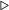Let V be the set of all double variables in the function
3: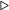Let v.D be the set of all definitions of variable v
4: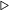Let d.state be the integerOkay state of variable v for definition d
5: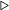Let v.U be the set of all uses of variable v
6: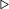Let u.state be the integerOkay state of variable v for use u
7:   for all v V do
8:   for all d v.D do
9:   d.state GETSTATEDEF(v,d)
10:   end for
11:   for all u v.U do
12:   u.state GETSTATEUSE(v,u)
13:   end for
14:   end for
15:  end procedure

16:  procedure GETSTATEDEF(v,d)
17: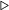Let v be a variable and d be a definition of v
18: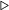Let d.deps be the dependency list of variable v for definition d
19: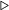Let d.RHS be the right hand side expression of definition d
20:
21: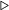if d is an assignment from a constant
22:   if ISCONSTASSIGNMENT(dthen
23:   if ISREALINTEGER(d.RHSthen
24: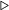if the RHS is a real integer
25:   return IntOk
26:   end if
27:   end if
28: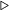if d is a copy statement
29:   if ISCOPYSTMT(dthen
30: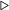add RHS variable (except v itself) to the dependency list of v for d
31:   d.deps d.depsd.RHS.varName
32:   d.deps d.deps-v
33:   return CondIntOk
34:   end if
35: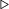if d is an assignment from a call to a builtin function
36:   if ISASSIGNMENTFROMBUILTIN(dthen
37: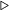if the RHS builtin always returns integer (eg. floor(), ceil(), etc.)
38:   if DOESBUILTINRETURNINT(d.RHSthen

39:   return IntOk
40:   end if
41: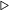if the RHS builtin always returns a double (eg. pi(), etc.)
42:   if DOESBUILTINRETURNDOUBLE(d.RHSthen
43:   return NotIntOk
44:   end if
45: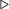if the builtin returns integer depending
46: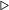on the type of input arguments (eg. plus(), minus(), etc.)
47:   if DOESBUILTINRETURNINTDEPENDS(d.RHSthen
48:add argumnets (except v) to the builtin to the dependency list of v for d
49:   d.deps d.depsd.RHS.args
50:   d.deps d.deps-v
51:   return CondIntOk
52:   end if
53:   return NotIntOk
54:   end if
55: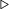if d is an assignment from an array access
56:   if ISASSIGNMENTFROMARRAYACCESS(dthen
57: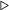add RHS array variable (except v itself) to the dependency list of v for d
58:   d.deps d.depsd.RHS.arrayName
59:   d.deps d.deps-v
60:   return CondIntOk
61:   end if
62: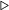default return NotIntOk
63:   return NotIntOk
64:  end procedure

65:  procedure GETSTATEUSE(v,u)
66: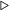Let v be a variable and u be a use expression of v
67: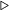Let u.deps be the dependency list of variable v for use u
68: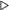if v is used as an array index in u
69:   if ISUSEARRAYINDEX(uthen
70:   return IntOk
71:   end if
72: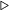if v is used as an argument to a builtin function
73:   if ISUSEBUILTINARGUMENT(uthen
74: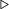if argument type does not affect the correctness of the result from builtin (eg. plus())
75:   if DOESARGTYPEAffECTRESULT(uthen

76:   return IntOk
77:   end if
78: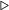if the argument type may affect the builtin result (eg. divide())
79:   if DOESARGTYPEAffECTRESULT(uthen
80:   return NotIntOk
81:   end if
82: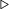if the affect of the argument type depends on the types of other arguments
83:   if DOESARGTYPEDEPENDONOTHERARGS(uthen
84:   u.deps u.depsu.args
85:   u.deps u.deps-v
86:   return CondIntOk
87:   end if
88:   end if
89: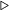if v is used in a copy statement
90:   if ISUSECOPYSTATEMENT(uthen
91:   return IntOk
92:   end if
93:   return NotIntOk
94:  end procedure

Algorithm 2: Fixed point solver for the IntegerOkay analysis
1:  procedure FIXEDPOINTSOLVER(V )
2: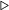Let V be the set of all the double variables in the function
3: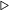Let Vbe the set of variables that can be declared as integers
4: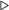Let v.D be the set of all definitions of variable v
5: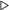Let d.state be the integerOkay state of variable v for definition d
6: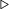Let v.U be the set of all uses of variable v
7: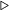Let u.state be the integerOkay state of variable v for use u
8: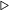Let v.State be the integerOkay state of variable v for the whole function
9: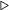Let v.State be initialized to ~
10:   V′←∅
11:   repeat
12:   Vold V
13:   for all v V do
14:   for all d v.D do
15:   v.State v.State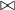d.state
16:   end for
17:   for all u v.U do
18:   v.State v.State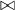u.state
19:   end for
20:   if v.State = IntOk then
21:   v′← v
22:   V′← V′∪v
23:   V V -v
24:   RESOLVEDEPENDENCIES(V,v)
25:   end if
26:   end for
27:   changed V′⁄= Vold
28:   until ¬changed
29:  end procedure

30:  procedure RESOLVEDEPENDENCIES(V,v)
31: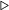Let V be the set of all the double variables in the function
32: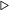Let vbe an IntegerOkay variable
33: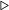Let v.D be the set of all definitions of variable v
34: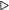Let d.state be the integerOkay state of variable v for definition d
35: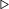Let d.deps be the dependency list of variable v for definition d
36: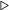Let v.U be the set of all uses of variable v
37: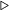Let u.state be the integerOkay state of variable v for use u

38: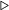Let u.deps be the dependency list of variable v for use u
39:   for all v V do
40:   for all d v.D do
41:   d.deps d.deps-v
42:   if d.deps =  then
43:   d.state IntOk
44:   end if
45:   end for
46:   for all u v.U do
47:   u.deps u.deps-v
48:   if u.deps =  then
49:   u.state IntOk
50:   end if
51:   end for
52:   end for
53:  end procedure

#### 8.4.2 STEP 2: The Fixed Point Solver

Once the initial state has been assigned for each use and each definition of every variable in STEP 1, the next step is to find the variables that can be of integer type across the function. The fixed point solver iteratively finds these variables until a fixed point is reached and no more variables are safe to be defined as integers.

The input to the fixed point solver is the set of all double variables V with state and dependency list initialized for each definition and each use of every variable v in V . The output of the fixed point solver is V, the set of variables that can be safely defined as integers. The fixed point solver also defines the variable v.State that stores the final state value for the variable v in the function, independent of any use or definition. For each variable v, v.State is assigned an initial empty value ~. Vis initially set to .

Algorithm 2 provides an algorithm for this fixed point solver. Note that the algorithm uses a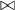operator to merge the state values of various definitions and uses of a variable to obtain its final state. Table 8.2 gives a definition of the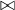operator used by this analysis. In the table, CondIntOk(s,s = ) is the case when d.depsu.deps = for a variable v, and CondIntOk(s,s) is the case when d.depsu.deps.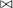IntOk CondIntOk(s,s = ∅) CondIntOk(s,s≠∅) NotIntOk ~ IntOk IntOk IntOk NotIntOk NotIntOk IntOk CondIntOk(s,s = ∅) IntOk IntOk NotIntOk NotIntOk CondIntOk(s,s = ∅) CondIntOk(s,s≠∅) NotIntOk NotIntOk NotIntOk NotIntOk CondIntOk(s,s≠∅) NotIntOk NotIntOk NotIntOk NotIntOk NotIntOk NotIntOk ~ IntOk CondIntOk(s,s = ∅) CondIntOk(s,s≠ ∅) NotIntOk ~

Table 8.2: Definition of themerge operator

### 8.5 An Example

Consider the following pseudocode for example:

/*1*/ x = 3.0;
/*2*/ y = 3.14;
/*3*/ z = x+y;
/*4*/ for (i = 0; i < 5; i++)
/*5*/   y = y+i;
/*6*/ end

In this example, the initialization step proceeds as follows. On line 1, x is IntegerOkay since 3.0 is a real integer. On line 2, y is Not IntegerOkay. On line 3, z is Conditionally IntegerOkay and depends on x and y, whereas x and y are IntegerOkay in their use in the expression x+y. On line 4, i is IntegerOkay in its definition i = 0, in its use in the expression i < 5, and also in the definition i++. On line 5, y is conditionally IntegerOkay and depends on i in its definition and it is IntegerOkay in its use in y+i. i is IntegerOkay in its use in y+i. Note that on line 5, we do not include y in its own dependency list, since if we say, y is conditionally IntegerOkay and depends on y, it is safe to declare y as integer as long as it does not have any other dependencies and is IntegerOkay everywhere else in the function.

The fixed-point solver for this example proceeds as follows. We look for variables that are IntegerOkay at every point in the function. x and i are two such variables and we can declare them to be an integer. We also remove x from the dependency list of definition of z on line 3, and i from the dependency list of definition of y on line 5. Next, we search again and find that y is IntegerOkay in its use on line 3 and line 5, and also in its definition on line 5, however it is Not IntegerOkay in its definition on line 2 and thus it cannot be declared as an integer. z on line 3 is dependent on y and thus it can also not be declared as an integer. At this point, we have reached a fixed point since there are no more upgrades. Finally, we declare x and i as integers, and y and z as doubles.

Chapter 9

Evaluation

___________________________________________________________________________________

In this chapter we evaluate the performance of our compiler. In this research our main aim was to generate X10 code for MATLAB such that its sequential performance would be comparable to the performance provided by the state of the art tools which translate MATLAB to more traditional imperative languages such as C and Fortran. To demonstrate our results, we compiled a set of 17 MATLAB programs to X10 via the MIX10 compiler and compared their performance results with those of the original MATLAB programs, C programs generated for our benchmarks via the MATLAB coder, and Fortran programs generated by the MC2FOR compiler.1 1We also compared our results to Octave, a widely used open source alternative to MATLAB. However, since Octave involves an interpreter, it performed slower than the standard MATLAB compiler (with a mean slowdown of 66.67 times slower) over all of our benchmarks, thus in this section we do not concentrate on comparison of our results with Octave. In addition to showing our best overall sequential performance, we also demonstrate the results of compiling the generated X10 code to Java compared to C++, effects on the performance for the various efficiency enhancing techniques discussed in this thesis, and finally the performance of the parallel X10 code generated for MATLAB parfor loops.

### 9.1 Benchmarks

The set of benchmarks used for our experiments consists of benchmarks from various sources; Most of them are from related projects like FALCON [RP99] and OTTER [QMSZ98a], Chalmers university of Technology2 2http://www.elmagn.chalmers.se/courses/CEM/ , “Mathworks’ central file excahange”3 3http://www.mathworks.com/matlabcentral/fileexchange , and the presentation on parallel programming in MATLAB by Burkardt and Cliff4 . This set of benchmarks covers the commonly used MATLAB features like arrays of different dimensions, loops, use of numerical functions like random number generation, trigonometric operations, and array operations like transpose and matrix multiplication. Table 9.1 gives a description of all the benchmarks we used and shows their special features.

 Benchmark Source Description Key features bbai MATLAB file exchange Implementation of the Babai estimation algorithm 2-D arrays, random number generation bubl McLab Bubble sort 1-D array, nested loops capr Chalmers University Computes the capacitance of a transmission line using finite difference and Gauss-Seidel method Array operations on 2-D arrays, nested loops clos Otter project Calculates the transitive closure of a directed graph Matrix multiplication, 2-D arrays crni Falcon project Crank-Nicholson solution to the heat equation read/write operations on a very large 2-D array dich Falcon project Dirichlet solution to Laplace’s equation Array operations on 2-D arrays, nested loops diff MATLAB file exchange Calculates the diffraction pattern of monochromatic light 2-D arrays, Concatenation operations, complex numbers edit MATLAB file exchange Calculates the edit distance between two strings many 1-D arrays of characters fiff Falcon project Computes the finite difference solution to the wave equation Array operations on 2-D arrays, nested loops lgdr Calculates derivatives of Legendre polynomials Array transpose on row vectors mbrt MCFOR project Computes Mandelbrot sets Complex numbers, parfor loop nb1d Otter project Simulates the 1-dimensional n-body problem Column-vectors, nested loops, parfor loop matmul McLab naive matrix multiplication 2-D arrays, nested loops, parfor loop mcpi McLab Calculates π by the Monte Carlo method Scalar values, Random number generation, parfor loop numprime Burkardt and Cliff Simulates the sieve of Eratosthenes for calculating number of prime numbers less than a given number Scalar values, nested loops, parfor loop optstop Burkardt and Cliff Solution to the optimal stopping problem Row vectors, random number generation, parfor loop quadrature Burkardt and Cliff Simulates the quadrature approach for calculating integral of a function Scalar values, parfor loop

Table 9.1: Benchmarks

### 9.2 Experimental Setup

We used Mathworks’ MATLAB release R2013a to execute our benchmarks in MATLAB and MATLAB coder. We also executed them using the GNU Octave version 3.2.4. We compiled our benchmarks to Fortran using the MC2FOR compiler and compiled the generated Fortran code using the GCC 4.6.3 GFortran compiler with optimization level -O3. To compile the generated X10 code from our MIX10 compiler, we used X10 version 2.4.0. We used OpenJDK Java 1.7.0_51 to compile and run Java code generated by the X10 compiler, and GCC 4.6.4 g++ compiler to compile the C++ code generated by the X10 compiler. All the experiments were run on a machine with Intel(R) Core(TM) i7-3820 CPU @ 3.60GHz processor and 16 GB memory running GNU/Linux(3.8.0-35-generic #52-Ubuntu). For each benchmark, we used an input size to make the program run for approximately 20 seconds on the de facto MATLAB compiler. We used the same input sizes for compiling and running benchmarks via other compilers. We collected the execution times (averaged over five runs) for each benchmark and compared their speedups over Mathworks’ MATLAB runtimes (normalized to one).

### 9.3 X10 Compiler Variations

The MIX10 compiler compiles the source MATLAB code to X10 code, which is then compiled by the X10 compiler. The X10 compiler is also a source to source compiler that provides two backends, a C++ backend that generates C++ code and a Java backend that generates Java code, which are then compiled by their respective compilers to executable code. Both these backends provide a -NO_CHECKS switch that generates the C++/Java code that does not include dynamic array bounds checks, which are otherwise included by default. As we described in section 4.1.1, we altered the X10 compiler to use column-major array indexing. We always used the -O optimization flag for the X10 compiler for both the backends, with notable exceptions where the X10 optimizer generated code which interacted extremely negatively with the Java JIT, as discussed in section 9.5.1. For all of our experiments, we used our IntegerOkay analysis, except for the experiment which investigates the performance impact of this analysis. Our best results were obtained by compiling the generated X10 code with the C++ backend with -NO_CHECKS enabled, where the X10 code itself was generated by the MIX10 compiler with simple arrays and our IntegerOkay analysis enabled.

### 9.4 Overall MIX10 Performance

We compared the performance of the generated X10 code with that of the original MATLAB code run on Mathworks’ implementation of MATLAB. To compare against the state of the art static compilers, we also compared the performance of the MIX10 generated X10 code with the C code generated by MATLAB coder and the Fortran code generated by the MC2FOR compiler.Figure 9.1: Performance of MIX10 vs other state-of-the-art static compilers, reported as speedups relative to Mathworks’ MATLAB, higher is better.

Figure 9.1 shows the speedups and slowdowns for the code generated for our benchmarks by different compilers. For MIX10 we have included the results for the X10 code compiled by the X10 C++ backend compiled, once with -NO_CHECKS enabled and once with -NO_CHEKCS disabled. For Fortran we included the results for the code generated without bounds checks. C code from MATLAB coder was generated with default settings and includes bounds checks. We also calculated the geometric mean of speedups(slowdowns) for all the benchmarks for each compiler.

Overall, one can see that the static compilers all provide excellent speedups, often of at least an order of magnitude faster. Thus, for the kinds of benchmarks in our benchmark set, it would seem that tools like the MATLAB coder, MC2FOR, and our MIX10 tools are very useful. MIX10 outperforms MATLAB coder in 9 out of 17 benchmarks, when compared with the X10 version compiled with bounds checks, and 10 out of 17 benchmarks when compared with the version with no bounds checks. For Fortran, the generated X10 does better in 7 out of 17 benchmarks with no bounds checks, and 6 out of 17 benchmarks with bounds checks enabled. Note that MATLAB coder was not able to compile 1 of our benchmarks (diff ) due to the dynamic array growth involved in it; MIX10 supports dynamic array growth.

We achieved a mean speedup of 4.8 over MATLAB, for the X10 code with bounds checks, and 6.8 for the x10 code with no bounds checks. On the other hand, MATLAB coder gave a mean speedup of 6.3 and MC2FOR gave a mean speedup of 10.2. However, we noticed that our mean result was skewed due to two benchmarks for which the generated X10 performed very poorly compared to the generated C code. These benchmarks are clos and lgdr. In the following paragraphs we explain the reason for their poor performance. If we do not consider these two benchmarks, we get a mean speedup of 6.7 for the X10 code with bounds checks compared to 5.2 for the C code. For the X10 code compiled with no bounds checks we get a mean speedup of 9.3 compared to 11.6 for Fortran.

clos involves repeated calls to the builtin matrix multiplication operation for the 2-dimensional matrices. The generated C code from MATLAB coder uses highly optimized matrix multiplication libraries compared to the naive matrix multiplication implementation used by MIX10. Thus, MIX10 gets a speedup of 0.02 as compared to 1.05 for C. Note that the generated Fortran code is also slowed down (speedup of 0.04) due to the same reason. As a future work, We plan to replace our matrix multiplication implementation with calls to an optimized library function.

lgdr involves repeated transpose of a row vector to a column vector. MATLAB and Fortran, both being array languages are highly optimized for transpose operations. MIX10 currently uses a naive transpose algorithm which is not optimized. We did achieve a speedup of over 10 times compared to MATLAB but it is not as good as the speedups achieved by C (speedup of 505.0) and Fortran (speedup of 336.7). For transpose operation also, we plan to replace our current implementation with an optimized implementation or a call to an optimized library function.

Both of these examples show that in addition to generating good code, another important task is developing optimized X10 library routines for key array computations.

Other interesting numbers are shown by optstop, fiff, nb1d, and quadrature. optstop gave a speedup of just 1.5 even without bounds checks. It involves repeated random number generation, which our experiments showed to be slow for the X10 C++ backend compared to Fortran and even the Java backend. This problem is worse with C, due to which the C code from MATLAB coder gives a speedup of mere 0.6(slowdown). Fortran performs better with a speedup of 4.3. bbai shows a similar pattern due to the same reason. fiff is characterized by stencil operations in a loop on a 2-dimensional array. These operations are also optimized by array-based languages like Fortran and MATLAB. For nb1d, Fortran performs better due to the use of column vectors in the benchmark, which are represented as 2-dimensional arrays in X10 but in Fortran they are represented as 1-dimensional and are optimized. 2-dimensional arrays are not as fast in X10 as the 1-dimensional arrays. quadrature involves repeated arithmetic calculations on a range of numbers. We achieve a speedup of about 200 times compared to MATLAB, however it is slow compared to speedups of 3348 and 502 by C and Fortran respectively. We believe that MATLAB coder leverages partial evaluation for optimizing numerical methods’ implementations.

For most of the other benchmarks, we perform better or nearly equal to C and Fortran code. Despite the facts that: (1) the sequential core of X10 is a high-level object oriented language which is not specialized for array-based operations; and (2) Generating the executable binaries via MIX10 involves two levels of source-to-source compilations (MATLAB X10 C++); we have achieved performance comparable to C, the state of the art in statically compiled languages and Fortran, a statically compiled language highly specialized for arrays.

### 9.5 X10 C++ Backend vs. X10 Java Backend

The X10 compiler provides two backends, a C++ backend that compiles the X10 code to native binary via C++, and a Java backend that compiles the X10 code to JVM code via Java. We were interested to see how well these backends perform when used to compile the MIX10 generated code. Even though we did not expect Java code to perform as well as the C++ code, our aim was to make sure that we achieved good performance, significantly better than MATLAB, for the X10 Java backend. This would enable MATLAB programmers to use our MIX10 compiler to generate code that could be integrated into Java applications.

In this section we present the performance comparison of the MIX10 generated X10 code compiled by the X10 C++ backend with that compiled by the X10 Java backend. Columns 3 and 4 of the Table 9.2 show speedups for our benchmarks compiled with the X10 C++ backend without bounds checks, and with bounds checks respectively. Columns 5 and 6 show these values for compilation with the X10 Java backend without bounds checks, and with bounds checks respectively. We also show the geometric mean of the speedups for all the 4 cases.

 Benchmark Matlab C++ (no checks) C++ (checks) Java (no checks) Java (checks) bbai 1 4.9 4.9 11.3 10.7 bubl 1 15.8 10.8 7.5 7.5 capr 1 13.5 12.7 11.1 6.3 clos 1 0.03 0.02 0.02 0.002 crni 1 6.5 5.3 5.6 4 dich 1 7.6 7.3 7 1.6 diff 1 4.6 4.4 0.3 0.3 edit 1 29.7 19.4 22.1 20 fiff 1 9.8 3.5 2.1 1.4 lgdr 1 13.5 11.9 10.6 10.6 mbrt 1 6.5 6.5 0.3 0.3 nb1d 1 6.2 4.8 5.5 4.1 matmul 1 14.7 2.5 1.1 0.8 mcpi 1 3.3 2.5 2.9 3 numprime 1 7.6 6.8 6.5 6.4 optstop 1 1.5 1.2 1.8 1.4 quadrature 1 200.9 182.6 167.4 154.5 Geometric mean 1 6.8 4.8 3.4 2.4

Table 9.2: MIX10 performance comparison : X10 C++ backend vs. X10 Java backend, speedups relative to Mathworks’ MATLAB, higher is better

The mean speedups for the C++ backend are 6.8 and 4.8 respectively for the version with bounds checks switched off, and the version with bounds checks switched on, whereas for Java backend these values are 3.4 and 2.4 respectively. This is expected, given that C++ is compiled to native binary while Java is JIT compiled.

bbai and optstop are the two exceptions, where Java performs better than C++. For bbai, both the Java versions gave a speedup of over 10, whereas for C++, the speedup is under 5 for both the versions. For optstop, the difference is not large, with C++ speedup at 1.5 (1.2 for the bounds checks version) compared to 1.8 (1.4 for the bounds checks version) for the Java backend. bbai is slower with X10 C++ backend because it includes repeated calls to the X10’s Random.nextDouble() function to generate random numbers. We found it to be significantly slower in the C++ backend compared to the Java backend. We have reported our findings to the X10 development team and they have validated our findings. optstop is slower for the same reason : It also involves repeated random number generation. Note that for these two benchmarks, even the C code generated via MATLAB coder is slower than the C++ code, with speedups of 4.2 and 0.6 respectively for bbai and optstop.

Other interesting results are for the benchmarks diff, fiff, mbrt and matmul. For these benchmarks, results from the Java backend are significantly slower compared to the C++ backend. diff and mbrt involve operations on complex numbers. In the X10 C++ backend, complex numbers are stored as structs and are kept on the stack, whereas in the Java backend, they are stored as objects and reside in the heap storage. fiff and matmul are characterized by repeated array access and read/write operations on 2-dimensional arrays. For these benchmarks, the Java backend performs significantly slower compared to the C++ backend with no bounds checks(2.1 vs. 9.8 for fiff and 1.1 vs. 14.7 for matmul), however compared to the performance by the C++ beckend with bounds checks, it is not as slow(2.1 vs. 3.5 for fiff and 1.1 vs. 2.5 for matmul). The reason is that even with bounds checks turned off for the X10 to Java compiler, The Java compiler by default has bounds checks on. These checks have a significant effect on performance for 2-dimensional array operations.

#### 9.5.1 When Not to Use the X10 -O

One of the most surprising results in this set of experiments was the fact that we had to sometimes disable the X10 -O optimizer switch when using the X10 Java backend.. For the benchmarks capr and dich, in the case when X10 bounds checks are switched on, we found very pathological performance, with slowdowns of over 2 orders of magntitude, when the X10 compiler’s optimization switch(-O) was used.

We recorded running times of 785.3 seconds for capr compared to 3.2 seconds without the optimization, and 1558.9 seconds for dich compared to 12.7 seconds without the optimization. With the help of the X10 development team we determined that switching on the optimization triggered code inlining for the array bounds check code, which then caused the resultant Java program to be too large to be handled by the JIT compiler. In fact, the Java JIT effectively gives up on this code and reverts to the interpreter.

Thus, it would seem that the X10 optimizer needs to be improved in order to apply aggressive inlining only when it does not have a negative impact on code size, and that different inlining strategies are needed for the C++ and Java backends.

### 9.6 Simple vs. Region Arrays

One of the key optimizations used by MIX10 is to use simple arrays, wherever possible, for higher performance. In this section we discuss the performance gains obtained by using simple arrays over region arrays and the specialized region arrays. A description of these three kinds of arrays provided by X10 was given in Sec. 2.1.3. Table 9.3 shows the relative speedups and slowdowns for our benchmarks compiled to use different kinds of X10 arrays for the C++ backend and the Java backend.

 X10 C++ backend X10 Java backend Benchmark Matlab Simple arrays Region arrays Sp. region arrays Simple arrays Region arrays Sp. region arrays bbai 1.0 4.9 2.7 2.7 11.3 6.4 6.6 bubl 1.0 15.8 11.4 11.6 7.5 3.6 3.7 capr 1.0 13.5 11.6 12.4 11.1 0.02 10.5 clos 1.0 0.03 0.01 0.01 0.02 0.01 0.01 crni 1.0 6.5 3.6 3.9 5.6 4 4.1 dich 1.0 7.6 6.7 7.0 7.0 0.01 0.02 diff 1.0 4.6 4.2 4.3 0.3 0.3 0.3 edit 1.0 29.7 4.3 3.6 22.1 9.4 9.4 fiff 1.0 9.8 2.0 2.8 2.1 1.4 1.4 lgdr 1.0 13.5 1.6 1.5 10.6 5.4 5.4 mbrt 1.0 6.5 6.3 6.5 0.3 5.1 5.1 nb1d 1.0 6.2 0.3 0.3 5.5 1.4 1.4 matmul 1.0 14.7 1.3 1.4 1.1 0.5 0.5 mcpi 1.0 3.3 2.8 2.7 2.9 3.0 3.0 numprime 1.0 7.6 5.7 5.7 6.5 6.3 6.3 optstop 1.0 1.5 0.4 0.4 1.8 1.2 1.3 quadrature 1.0 200.9 200.9 200.9 167.4 143.5 154.5 Geometric mean 1.0 6.8 2.7 2.8 3.4 1.3 1.9

Table 9.3: MIX10 performance comparison : Simple arrays vs. Region arrays vs. Specialized region arrays, speedup relative to Mathworks’ MATLAB, higher is better

For the C++ backend we obtained a mean speedup of 6.8 for Simple arrays, compared to 2.7 for region arrays and 2.8 for specialized region arrays. For the Java backend, we obtained speedups of 3.4, 1.3 and 1.9 for the simple arrays, region arrays, and the specialized region arrays respectively. These results are as expected in Sec. 4.1. For the C++ backend, most noticeable performance differences between simple arrays and region arrays are for edit, fiff, lgdr, nb1d, matmul and optstop. All of these benchmarks are characterized by large number of array accesses and read/write operations on 2-dimensional arrays, except optstop and edit, which have multiple large 1-dimensional arrays. The performance difference is most noticeable for nb1d, where the region arrays are about 20 times slower than the simple arrays. This is because nb1d involves simple operations on a large column vector. With simple arrays, since the compiler knows that it is a column vector, rather than a 2-dimensional matrix, even though it is declared as a 2-dimensional array, the performance can be optimized to match that of the underlying Rail. However, this is not possible for region arrays where the size of each dimension is not known statically. For the C++ backend, we do not observe significant performance differences between the region arrays and the specialized region arrays.

For the Java backend we observed a higher difference in the mean performance for the simple arrays and the region arrays. The mean speedup for region arrays is 1.3 whereas for simple arrays it is nearly 3 times more at 3.4. There is also a significant difference between the performance of specialized region arrays and region arrays. Speedup for specialized region arrays is 1.9. Like the C++ backend, here also, most noticeable performance gain for simple arrays is for benchmarks involving a large number of array accesses and read/writes. capr and dich ask for special consideration. For capr, even with X10 compiler’s bounds checks turned off, the region array version slows down by more than 500 times compared to the simple array version and even the specialized region array version. This again, is due to the fact that region arrays, with the dynamic shape checks, generated more code than the JIT compiler could handle. For dich the slowdown due to region arrays was about 700 times compared to simple arrays. For dich, even the specialization on region arrays was not enough to reduce the code size enough to be able to be JIT compiled.

### 9.7 Effect of the IntegerOkay Analysis

In this section we present an overview of the performance improvements achieved by MIX10 by using the IntegerOkay analysis. Table 9.4 shows a comparison of speedups gained by using the IntegerOkay analysis over those without using it. For this experiment we used results with X10 optimizations turned on and bounds checks turned off.

 X10 C++ backend X10 Java backend Benchmark Matlab IntegerOkay All Doubles IntegerOkay All Doubles bbai 1.0 4.9 3.8 11.3 8.2 bubl 1.0 15.8 2.2 7.5 4.2 capr 1.0 13.5 1.7 11.1 9.7 clos 1.0 0.03 0.02 0.02 0.01 crni 1.0 6.5 2.8 5.6 5.5 dich 1.0 7.6 1.0 7.0 5.8 diff 1.0 4.6 4.5 0.3 0.3 edit 1.0 29.7 13.5 22.1 20.0 fiff 1.0 9.8 1.0 2.1 1.8 lgdr 1.0 13.5 10.1 10.6 11.0 mbrt 1.0 6.5 6.1 0.3 0.3 nb1d 1.0 6.2 5.2 5.5 5.5 matmul 1.0 14.7 14.5 1.1 1.2 mcpi 1.0 3.3 3.3 2.9 2.9 numprime 1.0 7.6 7.6 6.5 7.1 optstop 1.0 1.5 1.0 1.8 1.0 quadrature 1.0 200.9 182.6 167.4 143.5 Geometric mean 1.0 6.8 3.4 3.4 2.9

Table 9.4: Performance evaluation for the IntegerOkay analysis, speedups relative to Mathworks’ MATLAB, higher is better

For the C++ backend, we observed a mean speedup of 6.8 which is two times the speedup gained by not using the IntegerOkay analysis, which is equal to 3.4. We observed a significant gain in performance by using IntegerOkay analysis for the benchmarks that involve significant number of array indexing operations. bubl, capr, crni, dich, and fiff show the most significant performance gains. The reason for this behaviour is that, X10 requires all array indices to be of type Long, thus if the variables used as array indices are declared to be of type Double (which is the default in MATLAB), they must be typecast to Long type. Double to Long is very time consuming because every cast involves a check on the value of the Double type variable to ensure that it can safely fit into Long type.

For the Java backend, with the IntegerOkay analysis, we get a mean speedup of 3.4 as compared to 2.9 without it. The reason for the lower difference as compared to that for the C++ backend is that, for Java backend, the X10 compiler does not generate the value checks for Double type values, instead it relies on the JVM to make these checks, resulting in a better performance. Also note that, without the IntegerOkay analysis, the C++ backend results are slower than the Java backend results for 9 out of 17 benchmarks.

To conclude, IntegerOkay analysis is very important for efficient performance of code involving Arrays, specially for the X10 C++ backend.

### 9.8 MATLAB parfor vs. MIX10 Parallel Code

Given that we have established that we can generate competitive sequential code, we wanted to also do a preliminary study to see if we could get additional benefits from the high-perfomance nature of X10. In this section we present the preliminary results for the compilation of MATLAB parfor construct to the parallel X10 code. 7 out of our 17 benchmarks could be safely modified to use the parfor loop. We compare the performance of the generated parallel X10 programs for these benchmarks to that of MATLAB code using parfor, and to their sequential X10 versions. For this experiment, we used the sequential versions of the generated X10 programs with optimizations and no bounds checks. For the parallel versions we used both the variants, with optimizations and bounds checks, and with optimizations and without bounds checks. It is important to note that the Intel(R) Core(TM) i7-3820 CPU used for this experiment has 4 CPU cores and 8 hardware threads, thus bounding the X10 parallelization speedup at 8. Table 9.5 shows the results for both the X10 C++ and the X10 Java backends.

 X10 C++ backend X10 Java backend Sequential Parallel Sequential Parallel Benchmarks MATLAB MATLAB parfor No checks No checks Checks No checks No checks Checks mbrt 1.0 1.7 6.5 25.3 25.3 0.3 1.3 1.3 nb1d 1.0 0.4 6.2 7.3 7.3 5.5 19.0 15.1 matmul 1.0 1.3 14.7 68.7 3.9 1.1 1.1 0.8 mcpi 1.0 4.6 3.3 15.7 15.9 2.9 18.1 18.2 numprime 1.0 5.3 7.6 30.0 30.9 6.5 24.8 26.4 optstop 1.0 4.1 1.4 2.0 2.1 1.8 10.7 9.7 quadrature 1.0 3.8 200.9 11.3 11.2 167.4 13.0 13.0 Geometric mean 1.0 2.3 8.9 14.5 9.8 3.7 7.8 7.1

Table 9.5: Performance evaluation for MIX10 generated parallel X10 code for the MATLAB parfor construct, speedups relative to Mathworks’ MATLAB, higher is better

For the X10 C++ backend we achieved a mean speedup of 14.5 for the generated parallel X10 code without bounds checks, which is around 7 times of the speedup for the MATLAB code with parfor, at a speedup of 2.3. Compared to X10 sequential code which has a mean speedup of 8.9, it is more than 1.5 times as fast. Even with the parallel X10 code with bounds checks we achieved a speedup of 9.8 which is over 4 times better than the MATLAB parfor code. The optstop benchmark is an interesting exception. It is actually slower (at a speedup of 2.1) than the MATLAB parfor version (at a speedup of 4.1). The sequential version of optstop is just slightly faster than the sequential MATLAB version, with a speedup of just 1.5 (due to the reasons explained earlier in Sec. 9.4) and the total time for the parallel execution of each iteration, and managing the parallelization of these activities is just slightly faster than the sequential version. We see a similar trend for the matmul benchmark for the X10 Java backend. It shows a speedup of just 0.8 (version with bounds checks) as compared to 1.3 for the MATLAB parfor version. For the parallel version of matmul, the speedup for the C++ backend with checks turned on is just 3.9 compared to 14.7 for the sequential version with no checks, however it is 1.6 times faster than the sequential version with checks, which has a speedup of just 2.5 (shown in figure 9.1). The quadrature benchmark shows very interesting numbers, with a slowdown of about 20 times and 13 times for the parallel X10 code compared to the sequential X10 code, for the C++ backend and the Java backend respectively. The quadrature benchmark consists of a loop with a large number of iterations (4600000 for our experiment), where each iteration performs an insignificant amount of computations with just 21 simple arithmetic operations on scalar values. A sequential speedup of over 200 times compared to the sequential MATLAB code indicates the insignificance of each iteration in terms of computation time. In the parallel X10 code, each iteration is executed as a separate activity, and the time taken to manage the large number of activities overshadows the sequential speedup. Also, each activity has an atomic statement, which is a blocking construct (when one activity is executing the atomic statement, all other activities are blocked) and adds further overhead to the activity management system. Performance numbers for quadrature assert the fact that not all parallel MATLAB programs are good candidates to be compiled to parallel X10 in a straight-forward fashion, specially the ones with large number of parallel loop iterations where each iteration itself does insignificant amount of work. As a future work, we plan to handle such programs with more sophisticated parallelization techniques. For example, one way to make a benchmark like quadrature more efficient would be to break the loop into two nested loops and executing the outer loop in a parallel fashion and the inner loop sequentially to ensure that each concurrent loop does substantial amount of work, however the challenge here is to determine the right number of iterations for the inner sequential loop to make it significant enough to take advantage of the parallelization.

Overall for the Java backend, we obtained a mean speedup of 7.8 for the X10 code with no bounds checks and 7.1 for the code with bounds checks. Compared to the MATLAB parfor version we obtained about 3.5 times better performance. The parallel X10 code is over 2 times faster than the sequential X10 code (speedup of 3.7). For both, the C++ backend, and the Java backend, the mean speedup for the sequential X10 code is also substantially faster than the MATLAB parallel code.

To conclude, the parallel X10 code provides much higher performance gains compared to the MATLAB parfor code and even the X10 sequential code, which by itself is most of the times faster than the MATLAB parallel code.

### 9.9 Summary

We showed that the MIX10 compiler with its efficient handling of array operations and optimizations like IntegerOkay can generate X10 code that provides performance comparable to the native code generated by the state of the art languages like C, which is fairly low-level, and Fortran, which itself is an array-based language. As a future work, we plan to use more efficient implementations of the builtin functions, and believe that it would further improve the performance of the code generated by MIX10.

With MIX10, we also took first steps to compile MATLAB to parallel X10 code to take full advantage of the high performance features of X10. Our preliminary results are very inspiring and we plan to continue in this direction further, in the future.

In addition to demonstrating that our approach leads to good code, these experiments have also been quite valuable for the X10 development team. Our generated code has stressed the X10 system more than the hand-written X10 benchmarks and has exposed places where further improvements can be made.

Chapter 10

Related Work

___________________________________________________________________________________

The work in this thesis presents a research compiler for MATLAB. We provide a source-to-source static compiler that targets the high-level, object oriented language, X10. Our approach is open and extensible and generates sequential X10 code that is competitive in performance to code in state-of-the-art languages like C and Fortran, generated by other MATLAB compilers. We also generated high performance code for MATLAB’s parfor loops, that showed great performance improvements.

### 10.1 Alternatives to MATLAB

Although our focus is on handling MATLAB itself, there are notable open source alternatives of MATLAB like Scilab[INR09], Julia[BKSE12], NumPy[Sci], FreeMat[DKB] and Octave[Oct]. Octave is an open source implementation of the MATLAB language and supports most of the MATLAB programs, however until the recently released version 3.8.1, Octave was solely an interpreted language and our experiments showed it to be orders of magnitude slower than MATLAB. We would like to evaluate its performance for the latest version that comes with a JIT compiler. Scilab and FreeMat are similar in syntax to MATLAB but have significant differences that prevent a significant portion of MATLAB programs to be able to run on these systems. NumPy is a python package for scientific computing, and Julia is a technical computing language. Julia also provides a fairly advanced distributed parallel execution environment. However, these alternative systems concentrate on providing open library support and have not tackled the problems of static compilation. We are investigating if there is any way of sharing some of their library support with MIX10. A Comparative evaluation of Matlab, Octave, FreeMat, Scilab, R, and IDL on a cluster computing system is presented in a technical report by Coman at al. [CBP+12] The MEGHA project[PAG11] provides an interesting approach to map MATLAB array operations to CPUs and GPUs, but supports only a very small subset of MATLAB.

### 10.2 Other MATLAB Compilers

There have been previous research projects on static compilation of MATLAB which focused particularly on the array-based subset of MATLAB and developed advanced static analyses for determining shapes and sizes of arrays. For example, FALCON [RP99] is a MATLAB to FORTRAN90 translator with sophisticated type inference algorithms. The McLab group has previously implemented a prototype Fortran 95 generator [Li09], and has more recently developed the next generation Fortran generator, MC2FOR [LH14] in parallel with the MIX10 project. Some of the solutions are shared between the projects, especially the parts which extend the Tamer. MATLAB Coder is a commercial compiler by MathWorks[Mata], which produces C code for a subset of MATLAB. We also compared MIX10’s performance to MATLAB coder. Below are more details about these and few other notable MATLAB compiler research projects:

• Falcon is a MATLAB-to-Fortran 90 compiler that applies type inference algorithms developed for the array programming language APL [JB01WS81Bud83] and set language SETL [Sch75]. It uses a static single-assignment(SSA) based intermediate representation, and inlines all functions and scripts into one large function. The Falcon project also curated a set of MATLAB benchmarks to measure the performance of their compiler. Several of our benchmarks are originally from Falcon’s benchmark suite.
• MCFOR is a prototype MATLAB-to-Fortran 95 generator developed by the McLAB research group and paved way for further research (of which MIX10 is a part) into developing efficient static MATLAB compilers for MATLAB. MC2FOR is the second generation MATLAB-to-Fortran 95 compiler built on top of the Tamer and MCSAF static analysis tools. MIX10 and MC2FOR share some of the solutions that extend the Tamer tool. We have also used MC2FOR for our performance evaluation. A good performance by both MIX10 and MC2FOR further validates the strength of our compilation techniques.
• MAJIC (MATLAB Just-In-time Compiler) [AP02] is a continuation of the Falcon project. It aims to exploit optimization opportunities in the following three areas1 1http://polaris.cs.uiuc.edu/majic/techniques.html : (1) source-level optimizations for matrix-based operations; (2) just-in-time compilation to defer compilation as much as possible and thus minimize the effects of challenges raised by MATLAB’s "wild" nature as we discussed in Chapter 6; and (3) specialized optimizations for operations on sparse matrices.
• MENHIR [CB98] is a retargetable MATLAB compiler that can generate C or Fortran code based on a target system description(MTSD). MTSD describes target system’s properties and implementation details and thus allows efficient code generation that exploits optimized sequential and parallel libraries.
• MATCH [Joi03] is a library based compilation environment for distributed heterogeneous adaptive computing systems consisting of field-programmable gate arrays and digital signal processors. It parallelizes MATLAB code based on user directives and generates C code that can call different runtime libraries.
• Otter [QMSZ98b] is a MATLAB to C compiler that targets parallel computers supporting parallel numerical libraries like ScaLAPACK [CDD+95]. It would be interesting, as a future work, to compare performance of the Otter compiler to that of MIX10 when it supports the parallel compilation of MATLAB more effectively.

A major difference between MIX10 and these compilers is that MIX10 targets an object oriented language with high-level array abstractions, and which is itself source-to-source compiled, whereas all the above mentioned projects either target JIT compilation or static compilation to either Fortran, which is itself an array based language and in fact was the precursor of MATLAB, or C, which is a fairly low-level language with advanced native compilers. Even though some of the techniques used by these compilers were helpful in generating X10 code for simple MATLAB constructs, the novelty of MIX10 lies in the fact that it identified major challenges involved in efficiently compiling MATLAB arrays and array based operations to X10 and achieved a performance comparable to that of compilers targeting C and Fortran.

### 10.3 Compilers Targeting X10

In terms of source-to-source compilers for X10, we are aware of two other projects. StreamX10 is a stream programming framework based on X10 [WTLY12]. StreamX10 consists of a stream programming language COStream and a compiler which translates programs in COStream to parallel X10 code. Tetsu discusses the design of a Ruby-based DSL for parallel programming that is compiled to X10 [Soh11]. Both these projects focus on compiling a parallel programming language to X10, a more efficient and generic parallel programming language. In the future, we would like to study these projects in more depth and hope to get useful insights into efficiently using X10 as a target language for parallelism in MATLAB. Chapter 11

Conclusions and Future Work

___________________________________________________________________________________

### 11.1 Conclusions

This thesis is about providing a bridge between two communities, the scientists/engineers/students who like to program in MATLAB on one side; and the programming language and compiler community who have designed elegant languages and powerful compiler techniques on the other side.

The X10 language has been designed to provide high-level array and concurrency abstractions, and our main goal was to develop a tool that would allow programmers to automatically convert their MATLAB code to efficient X10 code. In this way programmers can port their existing MATLAB code to X10, or continue to develop in MATLAB and use our MIX10 compiler as a backend to generate X10 code. Since X10 is publicly available under the Eclipse Public License (x10-lang.org/home/x10-license.html), users could have efficient high-performance code that they could freely distribute. Further, X10 itself can compile the code to either Java or C++, so our tool could be used in a tool chain to convert MATLAB to those languages as well.

Our tool is part of the McLab project, which is entirely open source. Thus, we are providing an infrastructure for other compiler researchers to build upon this work, or to use some of our approaches to handle other popular languages such as R.

In this thesis we demonstrated the end-to-end organization of the MIX10 tool, and we identified that the correct handling of arrays, the minimization of casting by safely mapping MATLAB double variables to X10 integer variables via IntegerOkay analysis, and handling concurrency features were the key challenges. We developed a custom version of X10’s rail-backed simple arrays, and identified where and how this could be used for generating efficient X10 code. For cases where precise static array shape and type information is not available, we showed how we can use the very flexible region-based arrays in X10, and our experiments demonstrated that it is very important to use the simple rail-backed arrays, for both the Java and C++ backends for X10.

Our experiments show that our generated X10 code is competitive with other state-of-the art code generators which target more traditional languages like C and Fortran. The C++ X10 backend produces faster code than the Java backend, but good performance is achieved in both cases.

One of the main motivations of choosing X10 as the target language is that it supports high-performance computing, which is often desirable for the computation-intensive applications developed by the engineers and scientists. To demonstrate how to take advantage of X10’s concurrency, we presented an effective translation of the MATLAB parfor construct to semantically equivalent X10.

Our experiments showed significant performance gains for our generated parallel X10 code, as compared to MATLAB’s parallel toolbox. This confirms that compiling MATLAB to a modern high-performance language can lead to significant performance improvements.

In the process of developing MIX10, we also stressed the X10 compiler with machine generated code and found it to be mostly robust and efficient. We found that the X10 Java backend’s optimizations may in some cases generate Java code that is too large to be JIT compiled. We also found the random number generation for the C++ backend to be slower than that for the Java backend. We reported our feedback to the X10 development team and hope that our findings would help to make X10 even better. Overall, we found that with the right techniques, X10 can be used as an effective and efficient target language for scientific and array-based languages, for both, the sequential core and the parallel part.

### 11.2 Future Work

Based on our positive experiences to date, we plan to continue improving the MIX10 tool. There are three major areas where we plan to take ahead the development of MIX10, with the aim of making it more complete and more efficient:

Exploiting parallelism
To help us better understand and efficiently exploit the inherent concurrency in the MATLAB programs, first of all we would like to thoroughly evaluate and fine tune the concurrency constructs we introduced in MATLAB via structured comments, and parallelism of the vectorized operations. Further, we would like to experiment to find the best way to tune the generated code for different sorts of parallel architectures. We are also planning to further our research into the direction of automatically parallelizing MATLAB codes through static analysis mixed with run-time checks.
Efficient builtin implementation
One of the greatest strengths of MATLAB is its  large and efficient set of builtin libraries. This thesis concentrates more on effective and efficient compilation of MATLAB constructs than finding the most efficient X10 implementations for the builtin operations. However, our experiments showed that there are some key library routines such as matrix multiply and transpose for which we need to have better X10 code for. Thus, there is scope for more work on X10 libraries, which could also be useful for other source-to-source compilers targeting X10.
Readability
The code that we generate is already quite clean, but has a quite low-level structure, which might not be easily readable for non-experienced X10 programmers. We would like to apply further transformations on it to aggregate some low-level expressions, and to make the generated code look as “natural" as possible. This would help MIX10 users, who are familiar with X10, to easily fine-tune the generated X10 code to better suite their execution environments. It would also help in easy maintenance of the generated X10 code, and better integration with hand-written X10 code.

Our tool is open source, and we hope that the other research teams will use our infrastructure, as well as learn from our experiences of generating effective and efficient X10 code.

Bibliography

___________________________________________________________________________________ [AP02] George Almási and David Padua. MaJIC: compiling MATLAB for speed and responsiveness. In PLDI ’02: Proceedings of the ACM SIGPLAN 2002 Conference on Programming language design and implementation, Berlin, Germany, 2002, pages 294–303. ACM. [BKSE12] Jeff Bezanson, Stefan Karpinski, Viral B. Shah, and Alan Edelman. Julia: A Fast Dynamic Language for Technical Computing. CoRR, abs/1209.5145, 2012. [Bud83] Timothy A. Budd. An APL compiler for the UNIX timesharing system. In APL ’83: Proceedings of the international conference on APL, Washington, D.C., United States, 1983, pages 205–209. ACM, New York, NY, USA. [CB98] Stéphane Chauveau and François Bodin. Menhir: An Environment for High Performance Matlab. In LCR ’98: Selected Papers from the 4th International Workshop on Languages, Compilers, and Run-Time Systems for Scalable Computers, 1998, pages 27–40. Springer-Verlag, London, UK. [CBP+12] E Coman, M Brewster, S Popuri, A Raim, and M Gobbert. A Comparative Evaluation of Matlab, Octave, FreeMat, Scilab, R, and IDL on Tara. Technical report, Baltimore County, US, 2012. [CDD+95] J. Choi, J. Demmel, I. Dhillon, J. Dongarra, S. Ostrouchov, A. Petitet, K. Staney, D. Walker, and R. C. Whaley. LAPACK Working Note 95: ScaLAPACK: A Portable Linear Algebra Library for Distributed Memory Computers – Design Issues and Performance. Technical report, Knoxville, TN, USA, 1995. [DH12a] Jesse Doherty and Laurie Hendren. McSAF: A static analysis framework for MATLAB. In Proceedings of ECOOP 2012, 2012, pages 132–155. [DH12b] Anton Dubrau and Laurie Hendren. Taming MATLAB. In Proceedings of OOPSLA 2012, 2012, pages 503–522. [DHR11] Jesse Doherty, Laurie Hendren, and Soroush Radpour. Kind analysis for MATLAB. In In Proceedings of OOPSLA 2011, 2011, pages 99–118. [DKB] Eugene Ingerman Demetrios Kyriakis and Samit Basu. Freemat. http://freemat.sourceforge.net/. [Doh11] Jesse Doherty. McSAF: An Extensible Static Analysis Framework for the MATLAB Language. Master’s thesis, McGill University, December 2011. [Dub12] Anton Dubrau. Taming matlab. Master’s thesis, April 2012. [EH04] Torbjörn Ekman and Görel Hedin. Reusable language specifications in JastAdd II. In Thomas Cleenewerck, editor, Evolution and Reuse of Language Specifications for DSLs (ERLS), 2004. Available from: http://prog.vub.ac.be/~thomas/ERLS/Ekman.pdf. [IBM12] IBM. X10 programming language. http://x10-lang.org, February 2012. [IBM13a] IBM. APGAS programming in X10 2.4, 2013. http://x10-lang.org/documentation/tutorials/apgas-programming-in-x10-24.html. [IBM13b] IBM. An introduction to X10, 2013. http://x10.sourceforge.net/documentation/intro/latest/html/node4.html. [INR09] INRIA. Scilab, 2009. http://www.scilab.org/platform/. [jas] JastAdd. http://jastadd.org/. [JB01] Pramod G. Joisha and Prithviraj Banerjee. Correctly detecting intrinsic type errors in typeless languages such as MATLAB. In APL ’01: Proceedings of the 2001 conference on APL, New Haven, Connecticut, 2001, pages 7–21. ACM, New York, NY, USA. [Joi03] Pramod G. Joisha. a MATLAB-to-C translator, 2003.http://www.ece.northwestern.edu/cpdc/pjoisha/mat2c/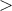. [LH14] Xu Li and Laurie Hendren. Mc2for: A tool for automatically translating matlab to fortran 95. In In Proceedings of CSMR-WCRE 2014, 2014, pages 234–243. [Li09] Jun Li. McFor: A MATLAB to FORTRAN 95 Compiler. Master’s thesis, McGill University, August 2009. [Mata] MathWorks. MATLAB Coder. http://www.mathworks.com/products/matlab-coder/. [Matb] Mathworks. Reduction variables. http://www.mathworks.com/help/distcomp/advanced-topics.html#bq_of7_-3. [Mat13] MathWorks. Parallel computing toolbox, 2013. http://www.mathworks.com/products/parallel-computing/. [Mol] Cleve Moler. The Growth of MATLAB and The MathWorks over Two Decades. http://www.mathworks.com/company/newsletters/news_notes/clevescorner/jan06.pdf. [Oct] GNU Octave. http://www.gnu.org/software/octave/index.html. [PAG11] Ashwin Prasad, Jayvant Anantpur, and R. Govindarajan. Automatic compilation of MATLAB programs for synergistic execution on heterogeneous processors. In Proceedings of the 32nd ACM SIGPLAN conference on Programming language design and implementation, San Jose, California, USA, 2011, PLDI ’11, pages 152–163. ACM, New York, NY, USA. [QMSZ98a]Michael J. Quinn, Alexey Malishevsky, Nagajagadeswar Seelam, and Yan Zhao. Preliminary Results from a Parallel MATLAB Compiler. In Proceedings of Int. Parallel Processing Symp., 1998, IPPS, pages 81–87. [QMSZ98b]Michael J. Quinn, Alexey Malishevsky, Nagajagadeswar Seelam, and Yan Zhao. Preliminary Results from a Parallel MATLAB Compiler. In Proc. Int. Parallel Processing Symp. (IPPS, 1998, pages 81–87. IEEE CS Press. [RP99] Luiz De Rose and David Padua. Techniques for the translation of MATLAB programs into Fortran 90. ACM Trans. Program. Lang. Syst., 21(2):286–323, 1999. [SBP+12] Vijay Saraswat, Bard Bloom, Igor Peshansky, Olivier Tardieu, and David Grove. X10 Language Specification, Version 2.2. January 2012. [Sch75] J. T. Schwartz. Automatic data structure choice in a language of very high level. Commun. ACM, 18(12):722–728, 1975. [Sci] Scipy.org. Numpy. http://www.numpy.org/. [Soh11] Tetsu Soh. Design and implementation of a DSL based on Ruby for parallel programming. Technical report, The University of Tokyo, January 2011. [The02] The Mathworks. Technology Backgrounder: Accelerating MATLAB, September 2002. http://www.mathworks.com/company/newsletters/digest/sept02/accel_matlab.pdf. [WS81] Zvi Weiss and Harry J. Saal. Compile time syntax analysis of APL programs. In APL ’81: Proceedings of the international conference on APL, San Francisco, California, United States, 1981, pages 313–320. ACM, New York, NY, USA. [WTLY12] Haitao Wei, Hong Tan, Xiaoxian Liu, and Junqing Yu. StreamX10: a stream programming framework on X10. In Proceedings of the 2012 ACM SIGPLAN X10 Workshop, Beijing, China, 2012, X10 ’12, pages 1:1–1:6. ACM, New York, NY, USA. Appendix A

XML structure for builtin framework

___________________________________________________________________________________

The below listing gives the XML structure for the builtin operation plus. It contains two upper level nodes for each real and complex numbers. Each of these have specialization nodes for the simple arrays of different dimensions, and the region arrays. Each of the specializations has 5 types of implementations.

1<plus>
2  <real>
3    <simple_array>
4      <array_1>
5         <type1>
6          {
7            val x: Double;
8            x = a+b;
9            return x;
10          }
11        </type1>
12        <type2>
13          {a.rank == b.rank}{
14            val x = new Array[Double](a.region);
15              for (p in a.indices()){
16                x(p) = a(p)+ b(p);
17              }
18              return x;
19          }
20        </type2>
21        <type3>
22          {
23            val x = new Array[Double](b.region);
24            for (p in b.region){
25              x(p) = a+ b(p);
26            }
27            return x;
28          }
29        </type3>
30        <type4>
31          {
32            val x = new Array[Double](a.region);
33            for (p in a.region){
34              x(p) = a(p)+ b;
35            }
36            return x;
37          }
38        </type4>
39        <type5>
40        </type5>
41      </array_1>
42      <array_2>
43        <type1>
44          {
45            val x: Double;
46            x = a+b;
47            return x;
48          }
49        </type1>
50        <type2>
51          {a.rank == b.rank}{
52            val x = new Array[Double](a.region);
53              for (p in a.indices()){
54                x(p) = a(p)+ b(p);
55              }
56              return x;
57          }
58        </type2>
59        <type3>
60          {
61            val x = new Array[Double](b.region);
62            for (p in b.region){
63              x(p) = a+ b(p);
64            }
65            return x;
66          }
67        </type3>
68        <type4>
69          {
70            val x = new Array[Double](a.region);
71            for (p in a.region){
72              x(p) = a(p)+ b;
73            }
74            return x;
75          }
76        </type4>
77        <type5>
78        </type5>
79      </array_2>
80      <array_3>
81        <type1>
82          {
83            val x: Double;
84            x = a+b;
85            return x;
86          }
87        </type1>
88        <type2>
89          {a.rank == b.rank}{
90            val x = new Array[Double](a.region);
91              for (p in a.indices()){
92                x(p) = a(p)+ b(p);
93              }
94              return x;
95          }
96        </type2>
97        <type3>
98          {
99            val x = new Array[Double](b.region);
100            for (p in b.region){
101              x(p) = a+ b(p);
102            }
103            return x;
104          }
105        </type3>
106        <type4>
107          {
108            val x = new Array[Double](a.region);
109            for (p in a.region){
110              x(p) = a(p)+ b;
111            }
112            return x;
113          }
114        </type4>
115        <type5>
116        </type5>
117      </array_3>
118    </simple_array>
119    <region_array>
120        <type1>
121          {
122            val x: Double;
123            x = a+b;
124            return x;
125          }
126        </type1>
127        <type2>
128          {a.rank == b.rank}{
129            val x = new Array[Double](a.region);
130              for (p in a.region){
131            x(p) = a(p)+ b(p);
132            }
133            return x;
134          }
135      </type2>
136      <type3>
137        {
138          val x = new Array[Double](b.region);
139          for (p in b.region){
140            x(p) = a+ b(p);
141          }
142          return x;
143        }
144      </type3>
145      <type4>
146        {
147          val x = new Array[Double](a.region);
148          for (p in a.region){
149            x(p) = a(p)+ b;
150          }
151          return x;
152        }
153      </type4>
154        <type5>
155        </type5>
156    </region_array>
157  </real>
158  <complex>
159    <simple_array>
160      <array_1>
161         <type1>
162          {
163            val x: Double;
164            x = a+b;
165            return x;
166          }
167        </type1>
168        <type2>
169          {a.rank == b.rank}{
170            val x = new Array[Double](a.region);
171              for (p in a.indices()){
172                x(p) = a(p)+ b(p);
173              }
174              return x;
175          }
176        </type2>
177        <type3>
178          {
179            val x = new Array[Double](b.region);
180            for (p in b.region){
181              x(p) = a+ b(p);
182            }
183            return x;
184          }
185        </type3>
186        <type4>
187          {
188            val x = new Array[Double](a.region);
189            for (p in a.region){
190              x(p) = a(p)+ b;
191            }
192            return x;
193          }
194        </type4>
195        <type5>
196        </type5>
197      </array_1>
198      <array_2>
199        <type1>
200          {
201            val x: Double;
202            x = a+b;
203            return x;
204          }
205        </type1>
206        <type2>
207          {a.rank == b.rank}{
208            val x = new Array[Double](a.region);
209              for (p in a.indices()){
210                x(p) = a(p)+ b(p);
211              }
212              return x;
213          }
214        </type2>
215        <type3>
216          {
217            val x = new Array[Double](b.region);
218            for (p in b.region){
219              x(p) = a+ b(p);
220            }
221            return x;
222          }
223        </type3>
224        <type4>
225          {
226            val x = new Array[Double](a.region);
227            for (p in a.region){
228              x(p) = a(p)+ b;
229            }
230            return x;
231          }
232        </type4>
233        <type5>
234        </type5>
235      </array_2>
236      <array_3>
237        <type1>
238          {
239            val x: Double;
240            x = a+b;
241            return x;
242          }
243        </type1>
244        <type2>
245          {a.rank == b.rank}{
246            val x = new Array[Double](a.region);
247              for (p in a.indices()){
248                x(p) = a(p)+ b(p);
249              }
250              return x;
251          }
252        </type2>
253        <type3>
254          {
255            val x = new Array[Double](b.region);
256            for (p in b.region){
257              x(p) = a+ b(p);
258            }
259            return x;
260          }
261        </type3>
262        <type4>
263          {
264            val x = new Array[Double](a.region);
265            for (p in a.region){
266              x(p) = a(p)+ b;
267            }
268            return x;
269          }
270        </type4>
271        <type5>
272        </type5>
273      </array_3>
274    </simple_array>
275    <region_array>
276        <type1>
277          {
278            val x: Double;
279            x = a+b;
280            return x;
281          }
282        </type1>
283        <type2>
284          {a.rank == b.rank}{
285            val x = new Array[Double](a.region);
286              for (p in a.region){
287            x(p) = a(p)+ b(p);
288            }
289            return x;
290          }
291      </type2>
292      <type3>
293        {
294          val x = new Array[Double](b.region);
295          for (p in b.region){
296            x(p) = a+ b(p);
297          }
298          return x;
299        }
300      </type3>
301      <type4>
302        {
303          val x = new Array[Double](a.region);
304          for (p in a.region){
305            x(p) = a(p)+ b;
306          }
307          return x;
308        }
309      </type4>
310        <type5>
311        </type5>
312    </region_array>
313  </complex>
314</plus>

Appendix B

MIX10 IR Grammar

___________________________________________________________________________________

Listing below gives the JastAdd implementation of the MIX10 IR grammar.

1Program ::= ClassBlock;
2PPHelper;
3ClassBlock ::= DeclStmt:Stmt* Method* <UseNewArray:Boolean>;
4abstract Stmt;
5CommentStmt:Stmt ::= <Comment:String>;
6BreakStmt:Stmt;
7ContinueStmt:Stmt;
8DeclStmt:Stmt ::= [MultiDeclLHS] LHS:IDInfo [RHS:Exp]
9<Mutable:Boolean> <Atomic:Boolean>;
10Literally:Stmt ::= <Verbatim:String>;
11LiterallyExp:Exp ::= <Verbatim:String>;
12BlockEnd:Literally;
13PointLooper:Stmt ::= PointID:IDUse ArrayID:IDUse
14  <DimNumber:int> [Min:Exp] [Max:Exp];
15Method ::= MethodHeader MethodBlock;
16MethodHeader ::= ReturnType:AccessVal <Name:String>
17  Args:IDInfo*;
18ReturnStmt:Stmt ::= ReturnVal:Exp*;
19Type:AccessVal ::= <Name:String>;
20MethodBlock:StmtBlock;
21AssignStmt:Stmt ::=  [MultiAssignLHS] LHS:IDInfo RHS:Exp
22  <TypeCast:Boolean> <Atomic:Boolean>;
23ExpStmt:Stmt ::= Exp;
24IDUse:Exp ::= <ID:String>;
25Dims ::= [Exp];
26IDInfo:Exp ::= Type <Name:String> <Shape:ArrayList>
27  <didShapeChange:Boolean> <isComplex:String> Value:Exp;
28MultiDeclLHS:Exp ::=  IDInfo* ;
29MultiAssignLHS:Exp ::=  IDInfo* ;
30abstract UnaryExp:Exp ::= Operand:Exp;
31PreIncExp:UnaryExp;
32PreDecExp:UnaryExp;
33MinusExp:UnaryExp;
34PlusExp:UnaryExp;
35NegExp:UnaryExp;
36EmptyExp:Exp;
37RegionBuilder:Exp ::= Lower:IDUse* Upper:IDUse*
38  ArrayID:IDUse;
39SimpleArrayExp:Exp ::= Values:Exp* Point* Type;
40Point:Exp ::= CoOrd:IntLiteral* ;
41ArrayAccess:AccessVal ::= ArrayID:IDUse Indices:Exp*
42  <IsColVector:Boolean>;
43ArraySetStmt:Stmt ::= LHS:IDInfo Indices:Exp* RHS:Exp;
44SubArraySetStmt:Stmt ::= LHS:IDInfo Lower:Exp* Upper:Exp*
45  RHS:Exp;
46SubArrayGetExp:Exp ::= Lower:Exp* Upper:Exp* ArrayID:IDUse;
47abstract LiteralExp:Exp;
48Literal:LiteralExp ::= <Literal:String>;
49StringLiteral:Literal;
50FPLiteral:Literal;
51DoubleLiteral:Literal;
52IntLiteral:Literal;
53BoolLiteral:Literal;
54LongLiteral:Literal;
55CharLiteral:Literal;
56abstract BinaryExp:Exp ::= LeftOp:Exp RightOp:Exp;
57abstract ArithExp:BinaryExp;
58abstract MultiplicativeExp:ArithExp;
59MulExp:MultiplicativeExp;
60DivExp:MultiplicativeExp;
61ModExp:MultiplicativeExp;
62abstract AdditiveExp:BinaryExp;
63AddExp:AdditiveExp;
64SubExp:AdditiveExp;
65IncExp:AdditiveExp;
66DecExp:AdditiveExp;
67abstract RelationalExp:BinaryExp;
68LTExp : RelationalExp ;
69GTExp : RelationalExp ;
70LEExp : RelationalExp ;
71GEExp : RelationalExp ;
72abstract EqualityExp : RelationalExp;
73EQExp : EqualityExp ;
74NEExp : EqualityExp ;
75abstract LogicalExp:BinaryExp;
76AndExp:LogicalExp;
77OrExp:LogicalExp;
78Modifiers ::= Modifier*;
79Modifier ::= <ID:String>;
80Identifier:AccessVal ::= <Name:String>;
81ForStmt:Stmt ::= <isParfor:Boolean> AssignStmt Condition:Exp
82  Stepper:AdditiveExp LoopBody Lower:IDUse Upper:IDUse
83  Incr:IDUse;
84WhileStmt:Stmt ::= Condition:Exp LoopBody;
85StmtBlock:Stmt ::= Stmt*;
86LoopBody:StmtBlock;
87IfElseStmt:Stmt ::= IfElseIf* [ElseBody];
88IfElseIf:Stmt ::= Condition:Exp IfBody;
89IfBody:StmtBlock;
90ElseBody:StmtBlock;
91abstract Exp;
92AtStmt:Stmt ::= Place:Exp AtBlock;
93AtBlock:StmtBlock;
94AsyncStmt:Stmt ::= AsyncBlock;
95AsyncBlock:StmtBlock;
96FinishStmt:Stmt ::= FinishBlock;
97FinishBlock:StmtBlock;
98AtomicStmt:Stmt ::= AtomicBlock;
99AtomicBlock:StmtBlock;
100WhenStmt:Stmt ::= Condition:Exp WhenBlock;
101WhenBlock:StmtBlock;
102abstract AccessVal:Exp;
103abstract MethodCall:Exp;
104BuiltinMethodCall:MethodCall ::= BuiltinMethodName:MethodId
105  Argument:Exp*;
106MethodId:AccessVal ::= <Name:String>;
107UserDefMethodCall:MethodCall ::= UserDefMethodName:MethodId
108  Argument:Exp*;